# Concise Selina Solutions for Class 9 Maths Chapter 16- Area Theorems

Selina solutions for Class 9 Maths Chapter 16 Area Theorems are provided here. The concepts which are taught in Class 9 are important to be understood as these concepts are continued in Class 10. To score good marks in Class 9 Mathematics examination, it is advised to solve questions provided in each exercise of all the chapters in the Selina book. These Selina solutions for Class 9 Maths help the students in understanding all the concepts in a better way. Download pdf of Class 9 Maths chapter 16 Selina Solutions from the given links.

### Download PDF of Selina Solutions for Class 9 Maths Chapter 16:-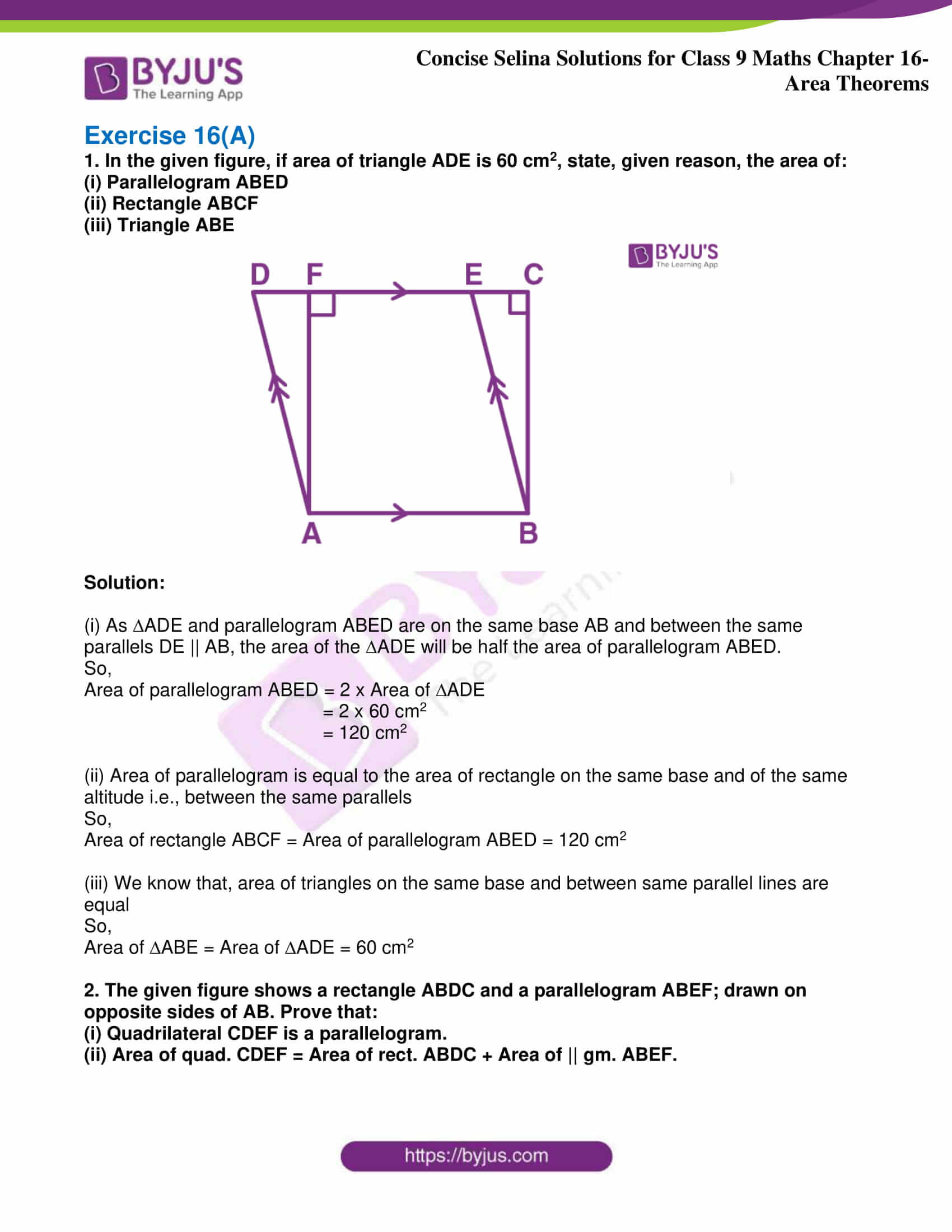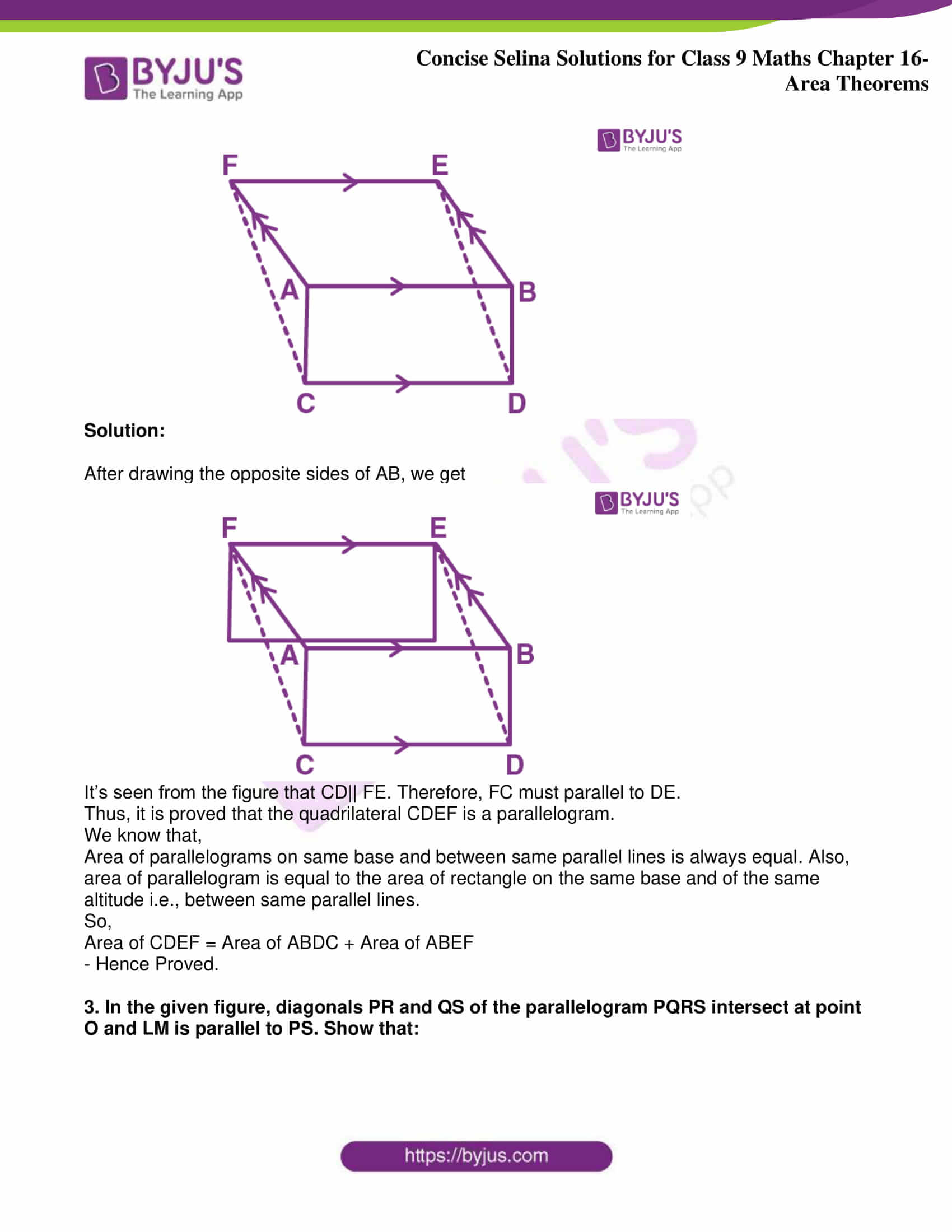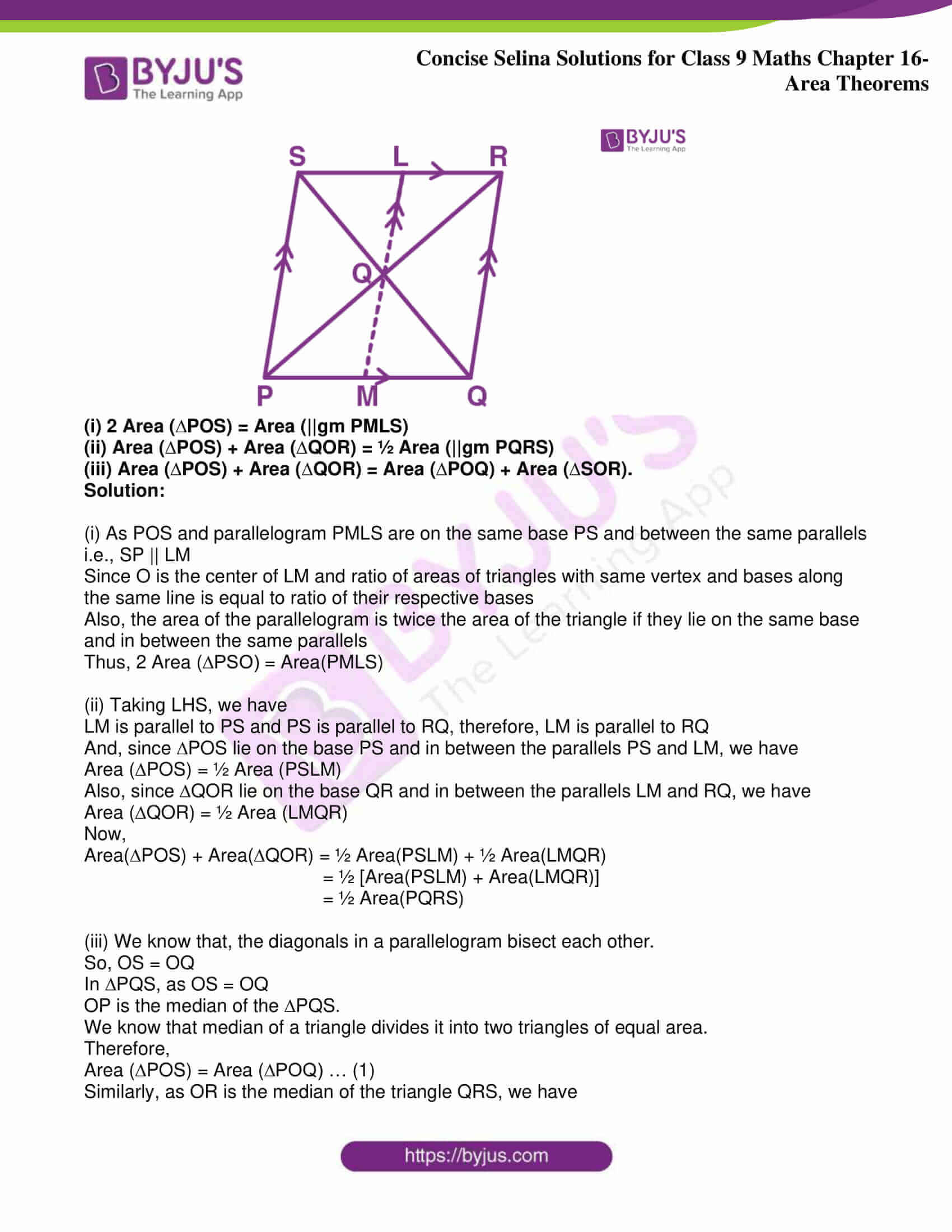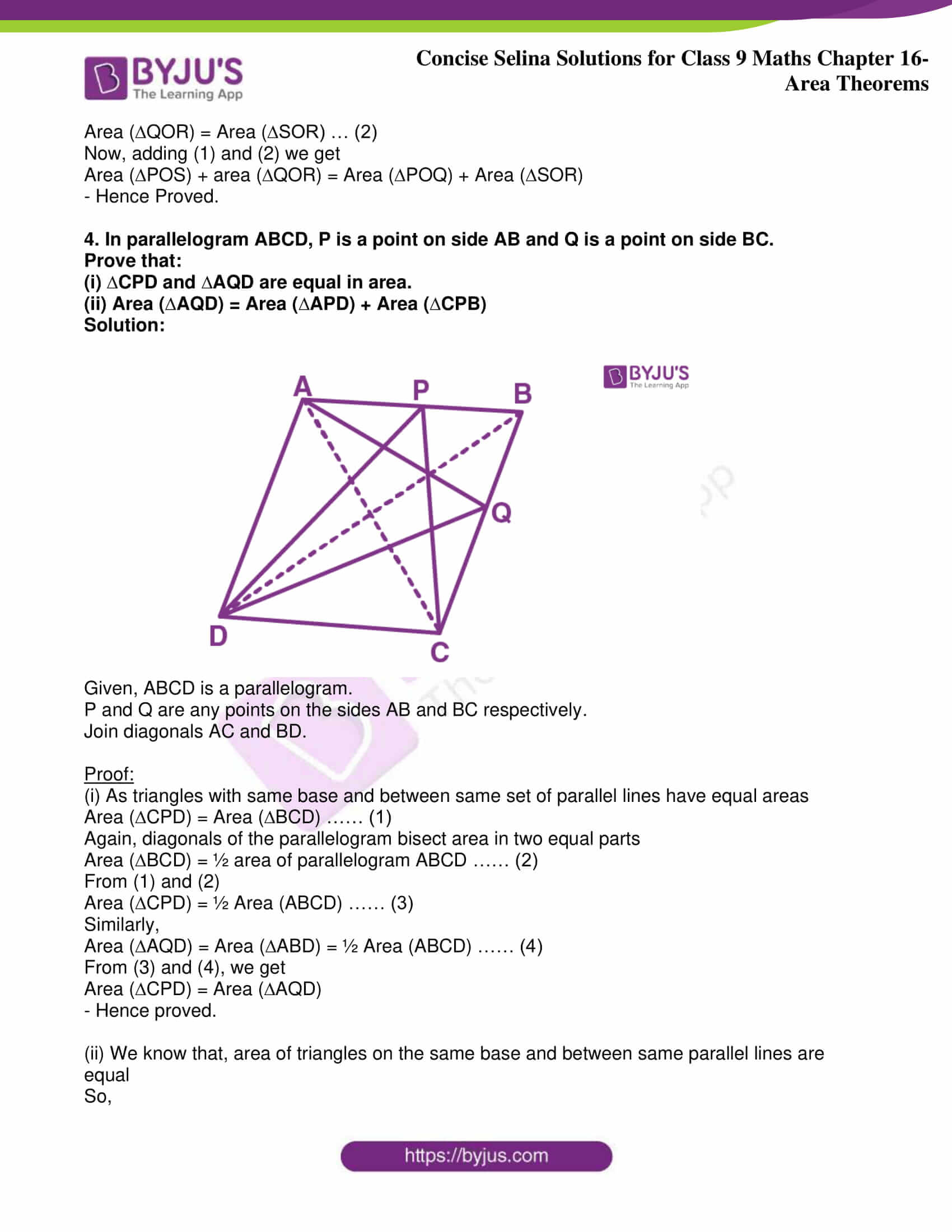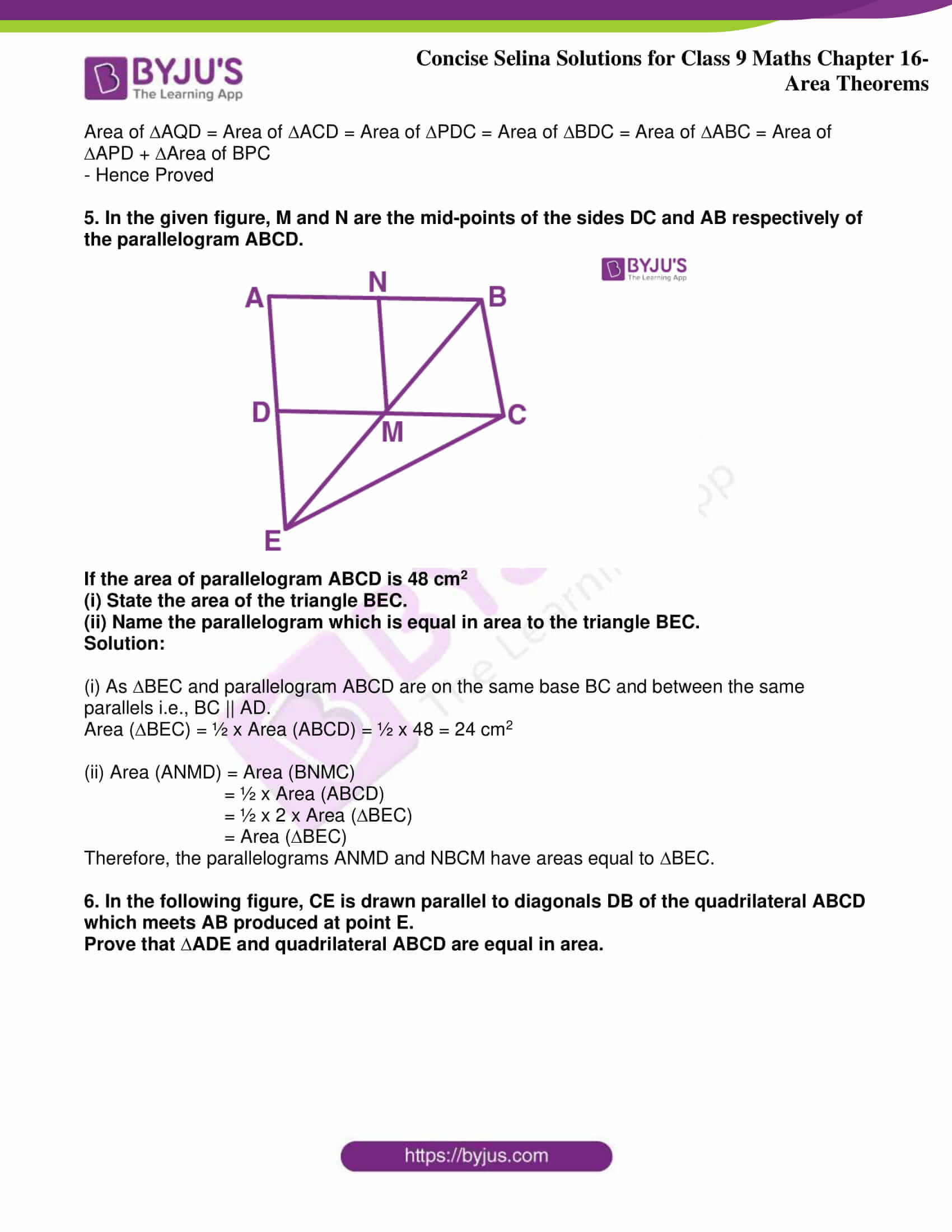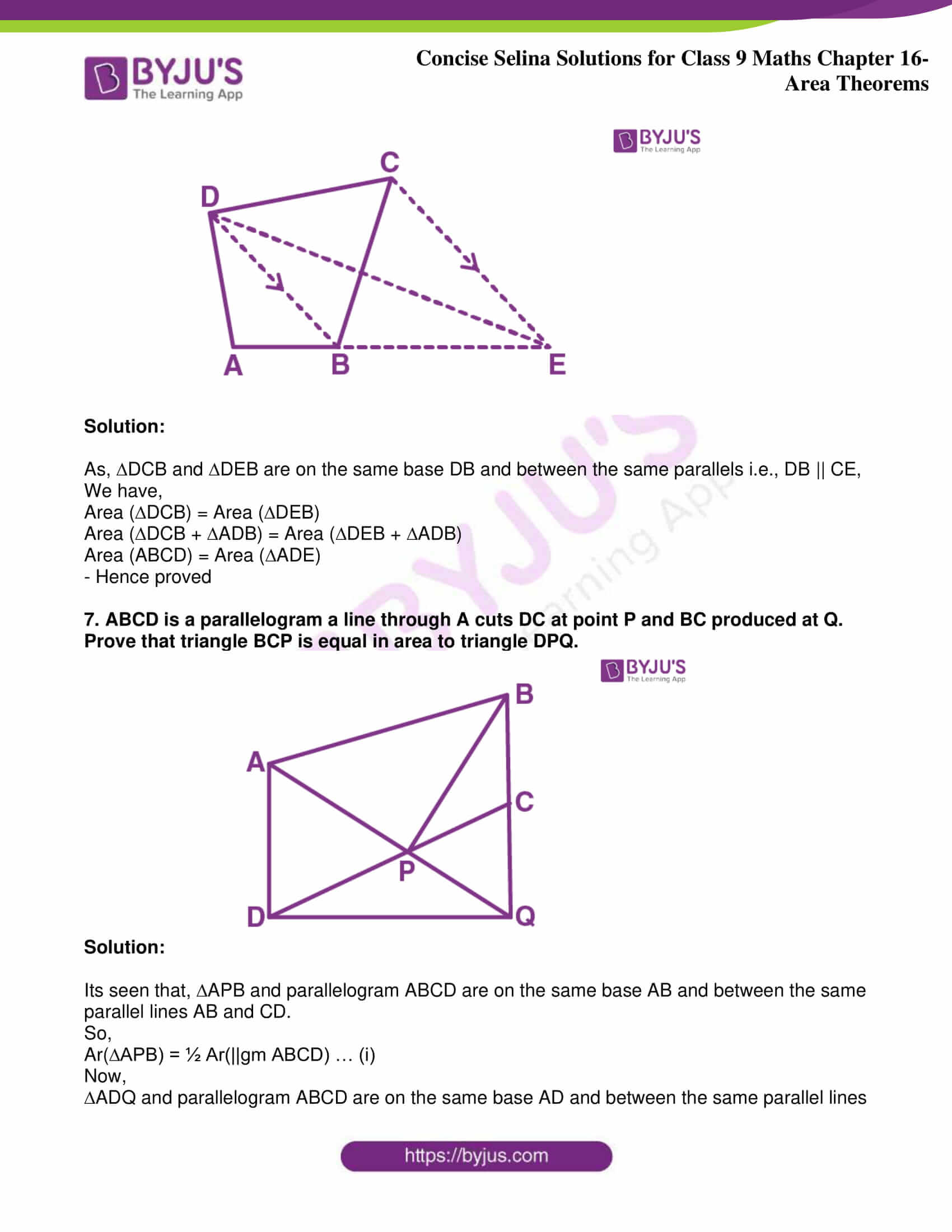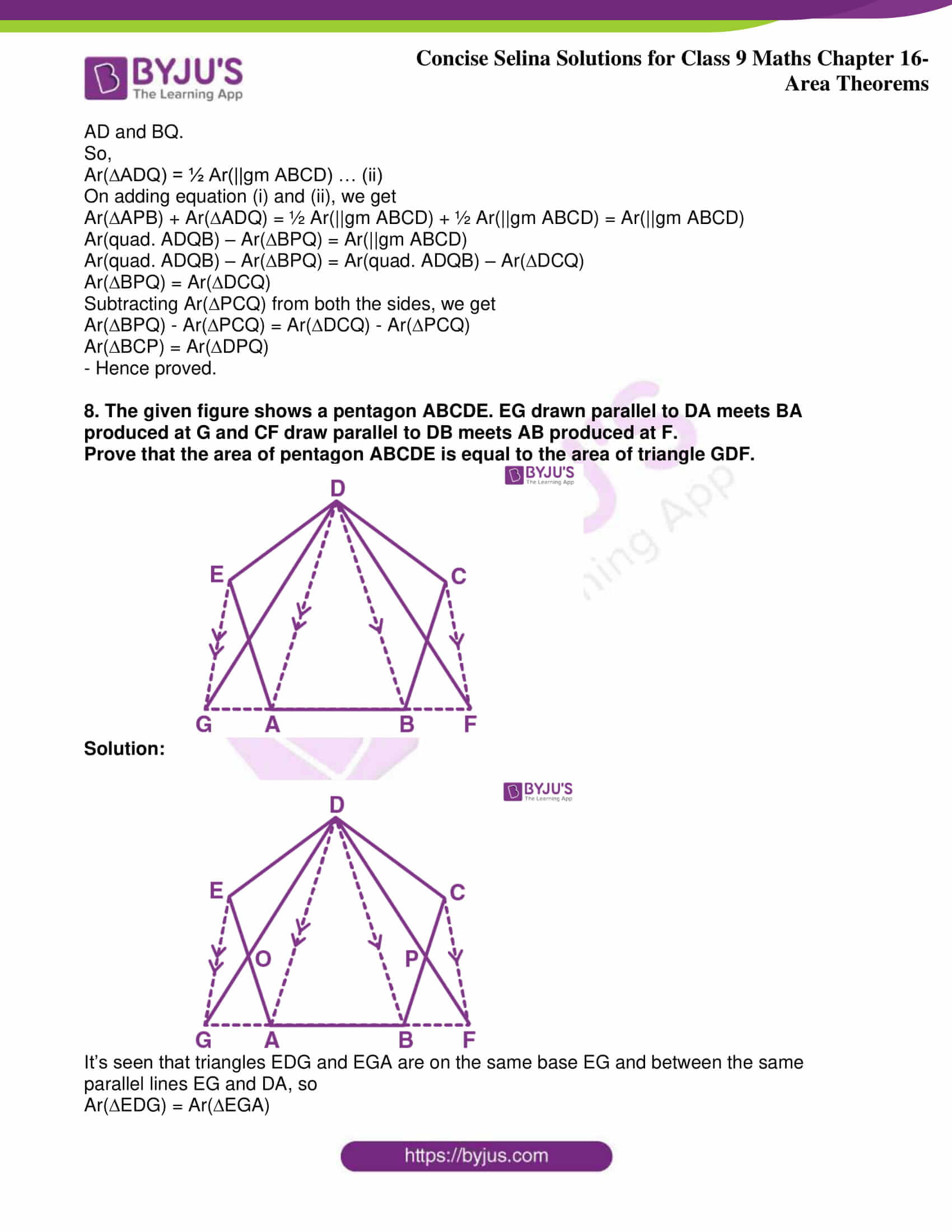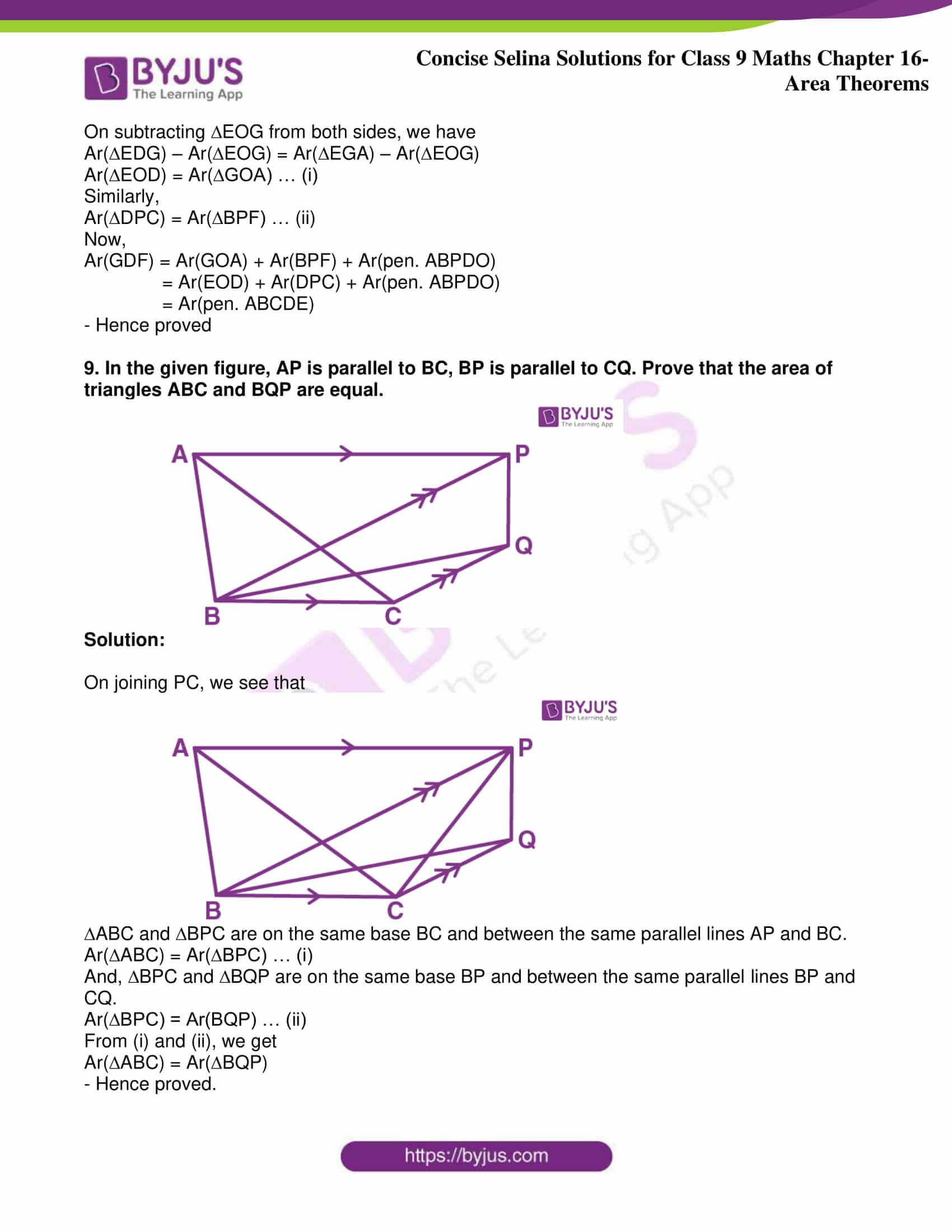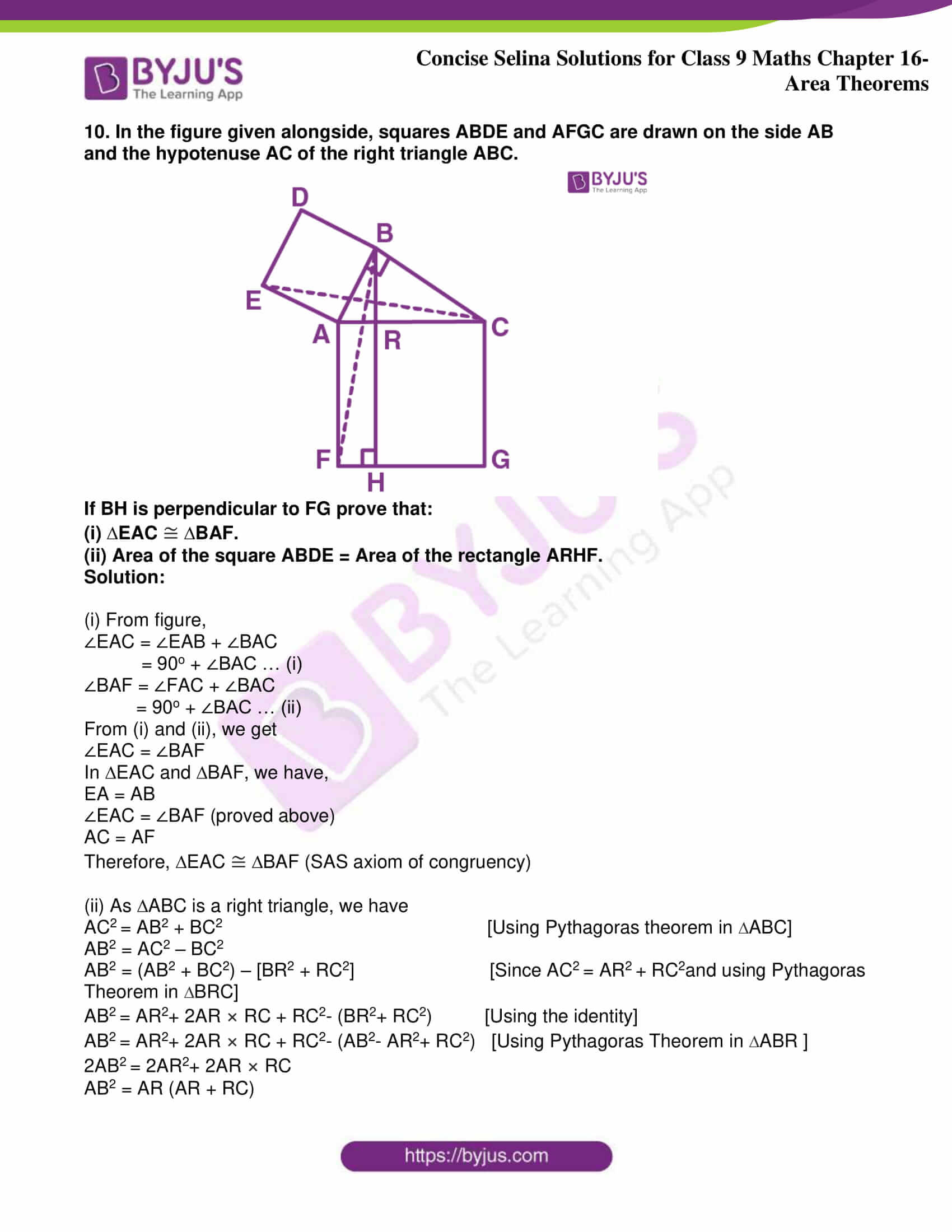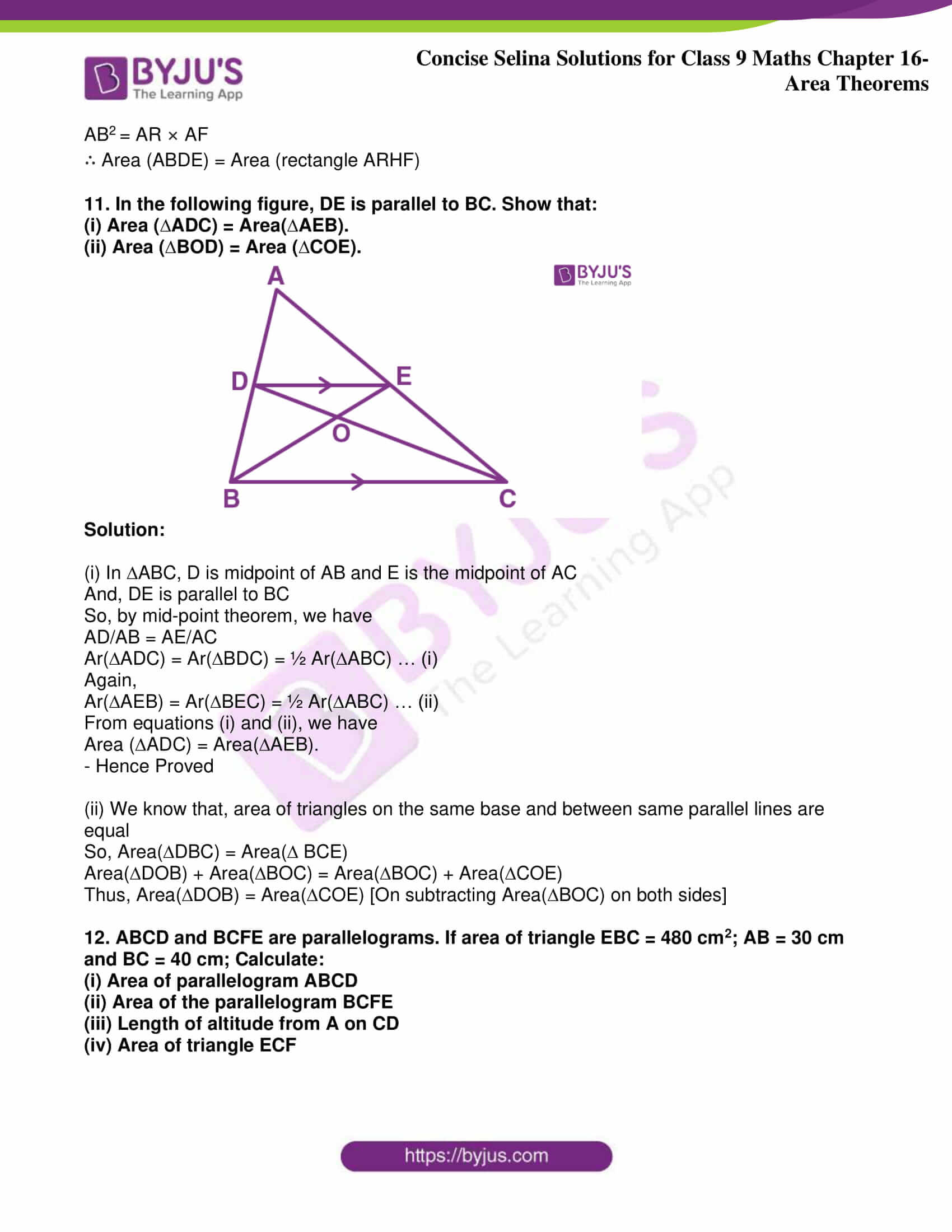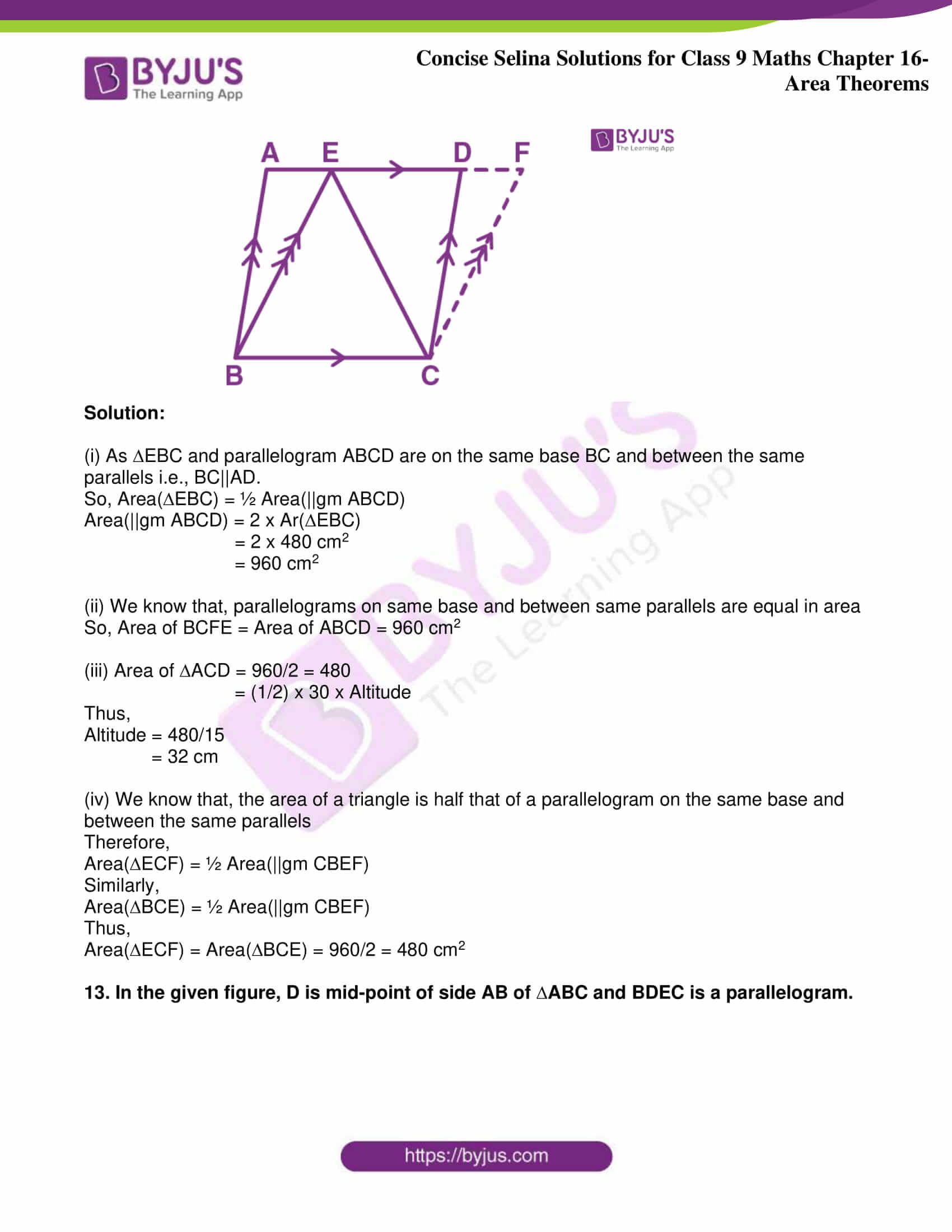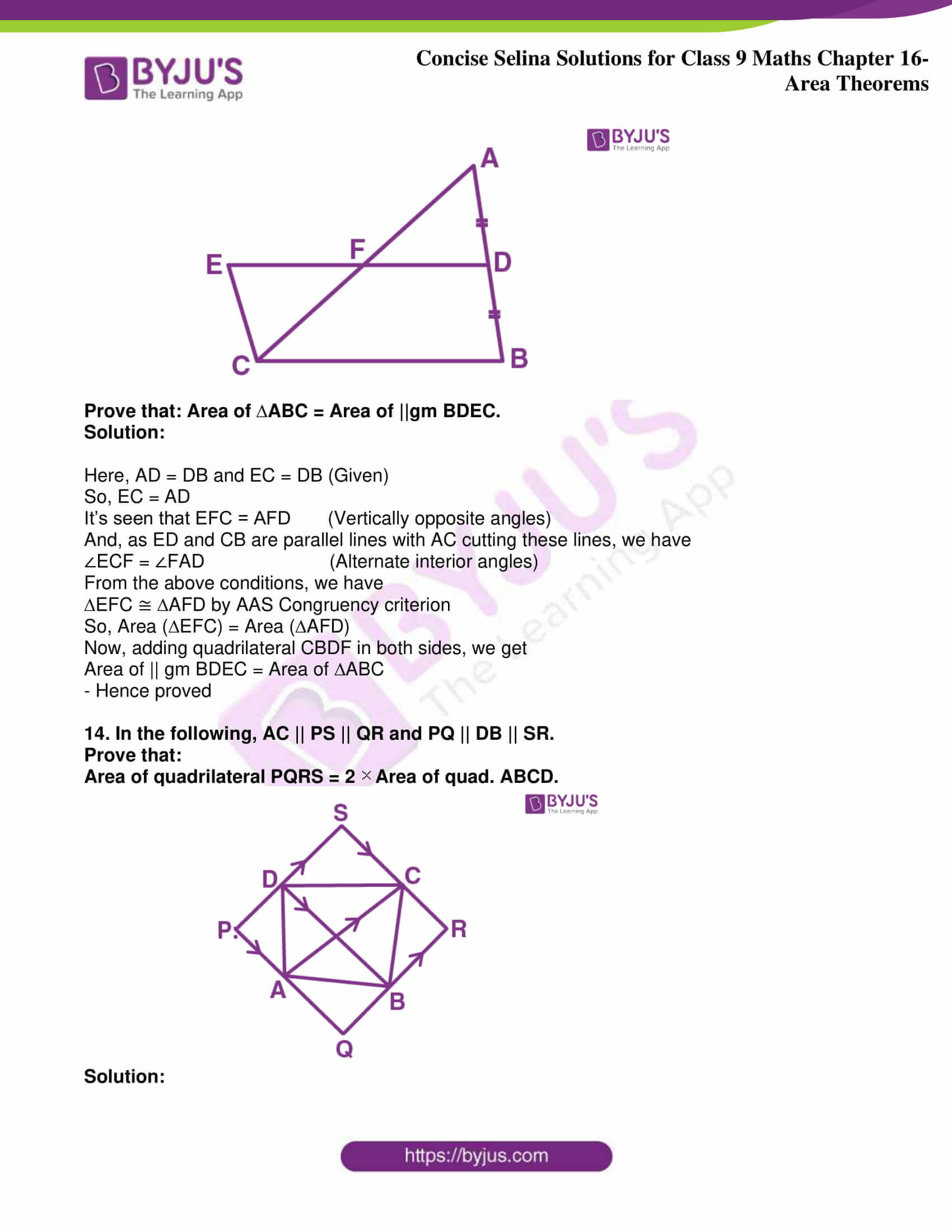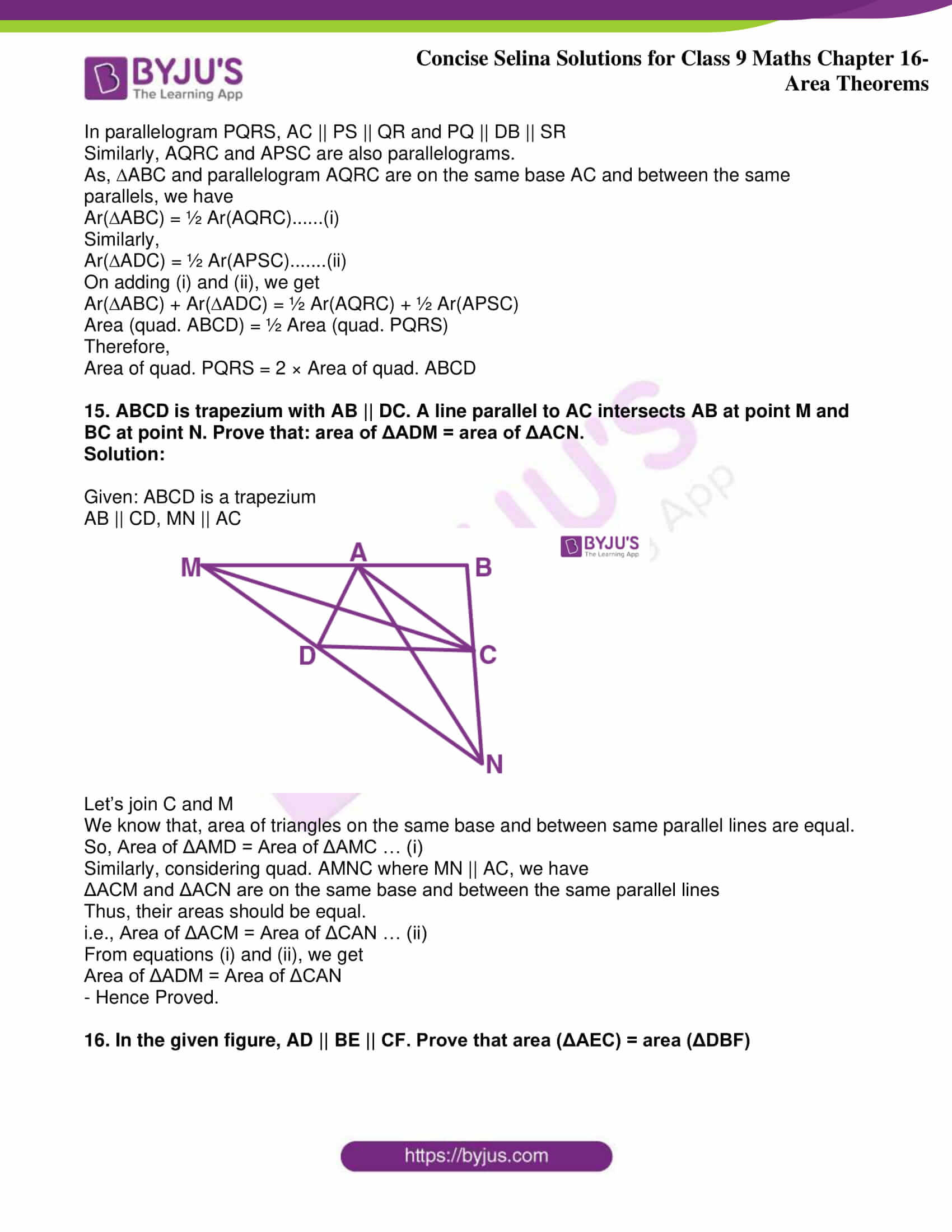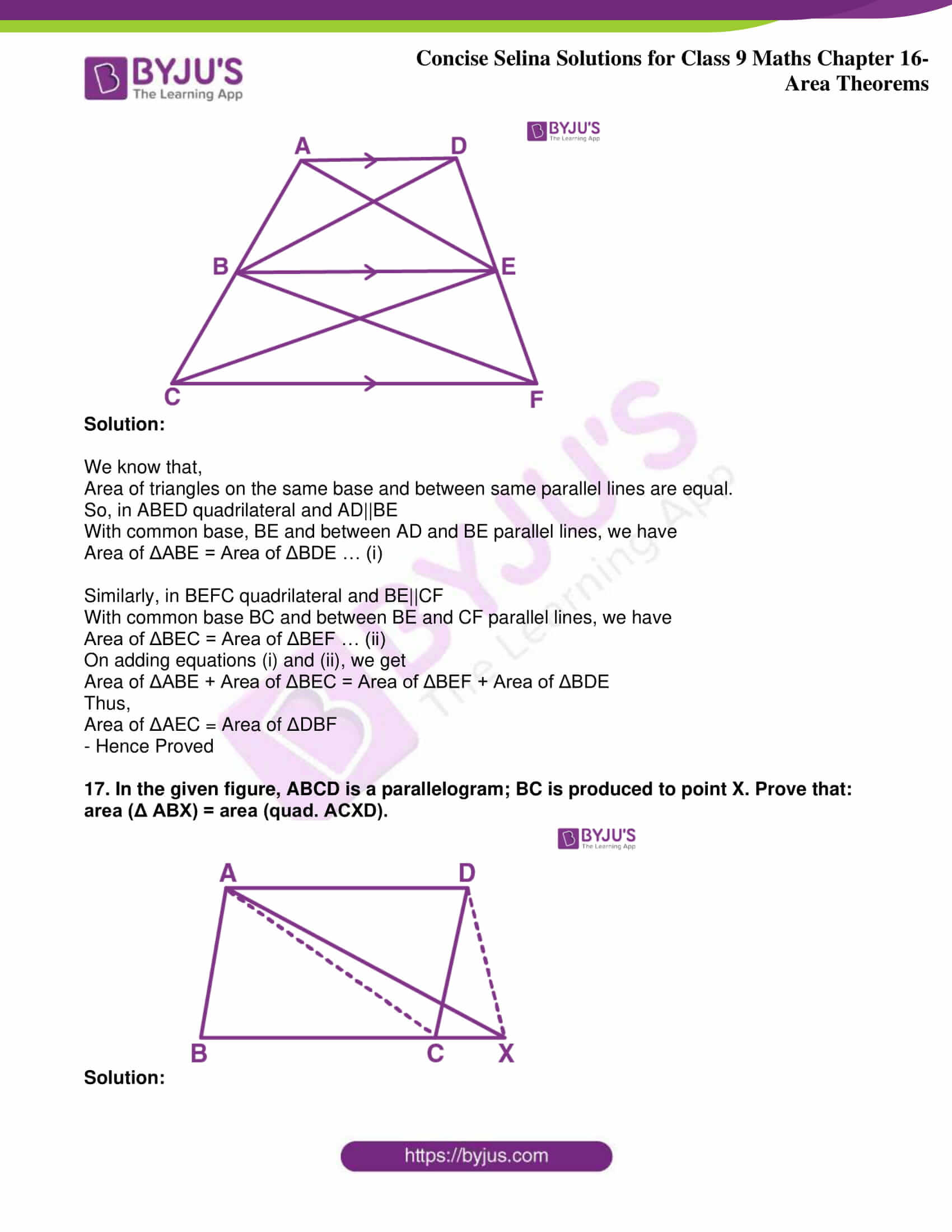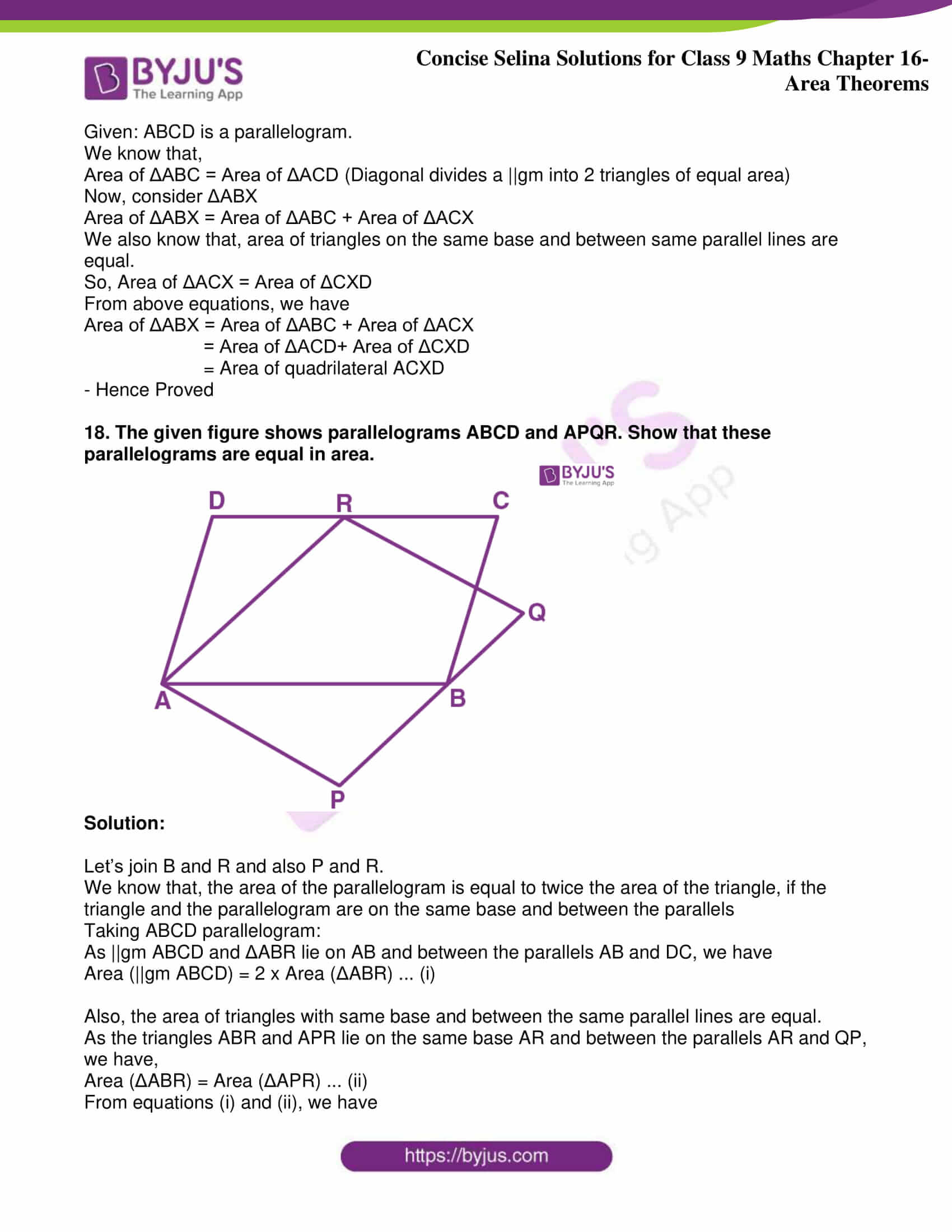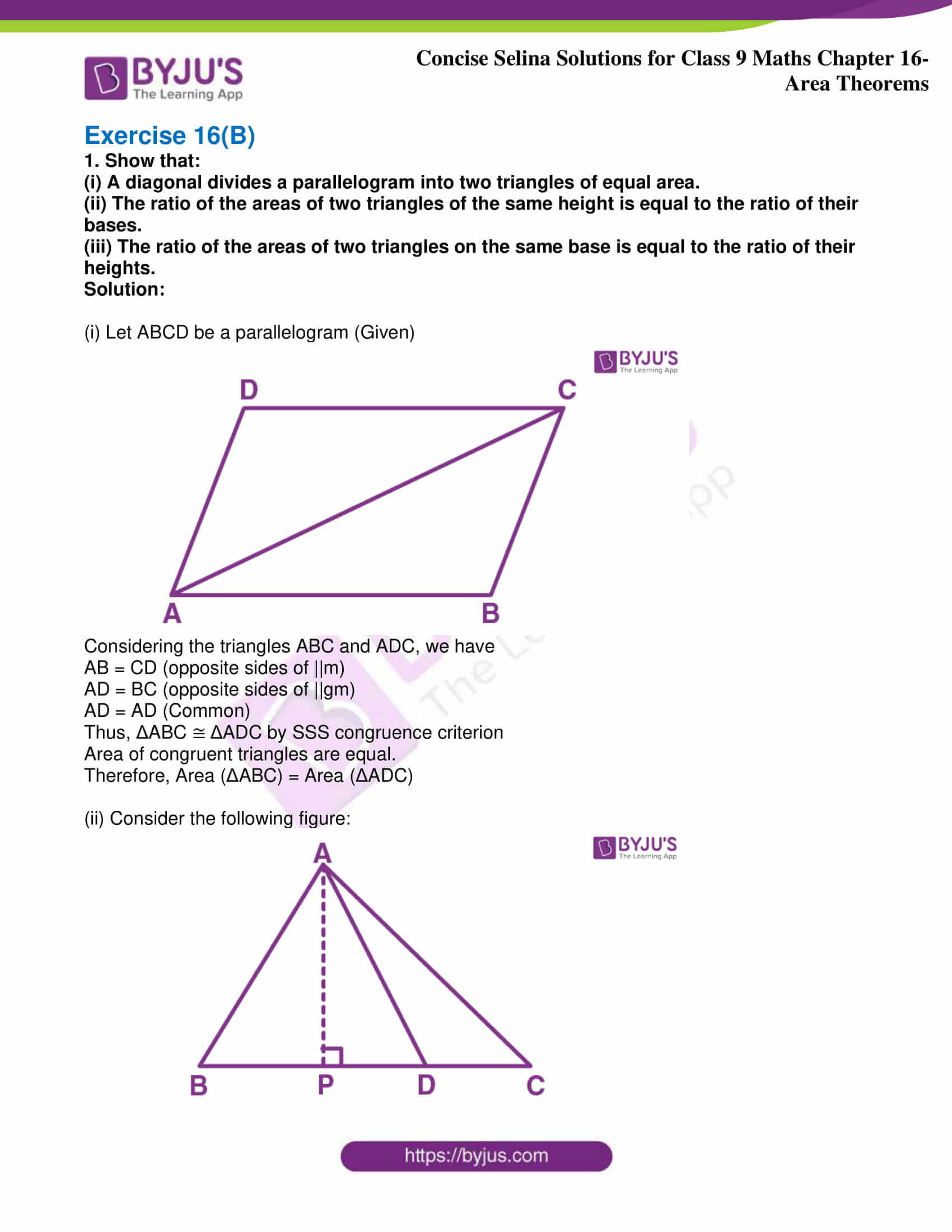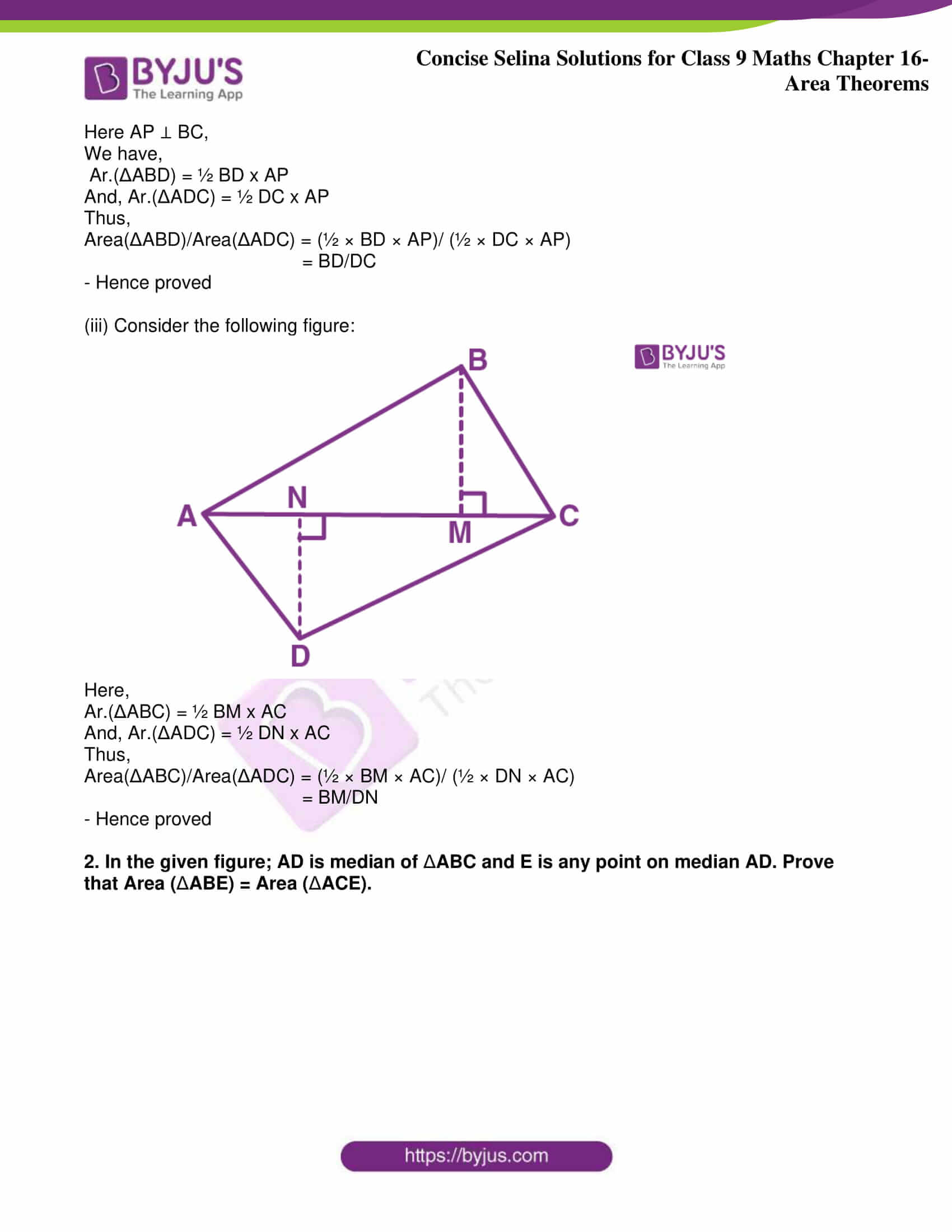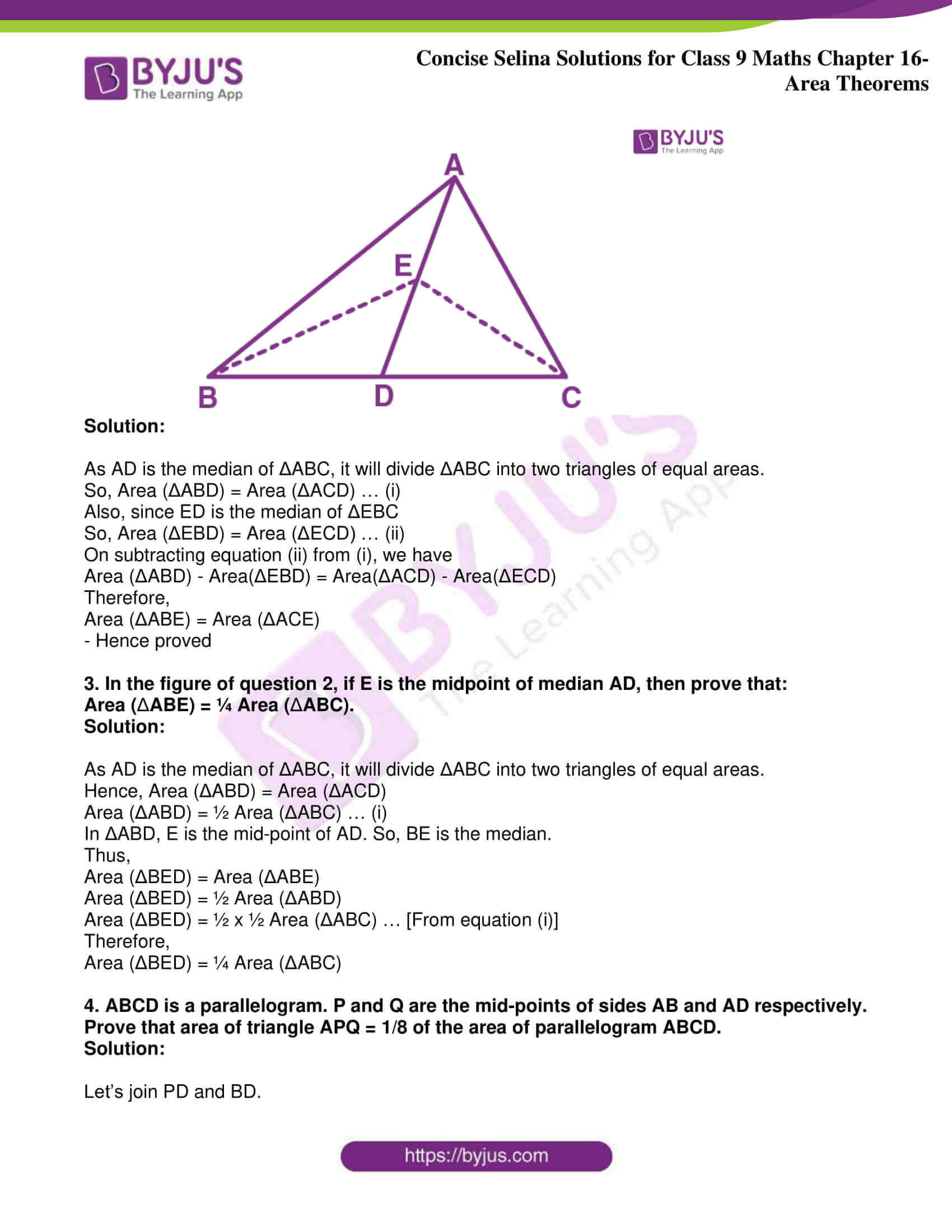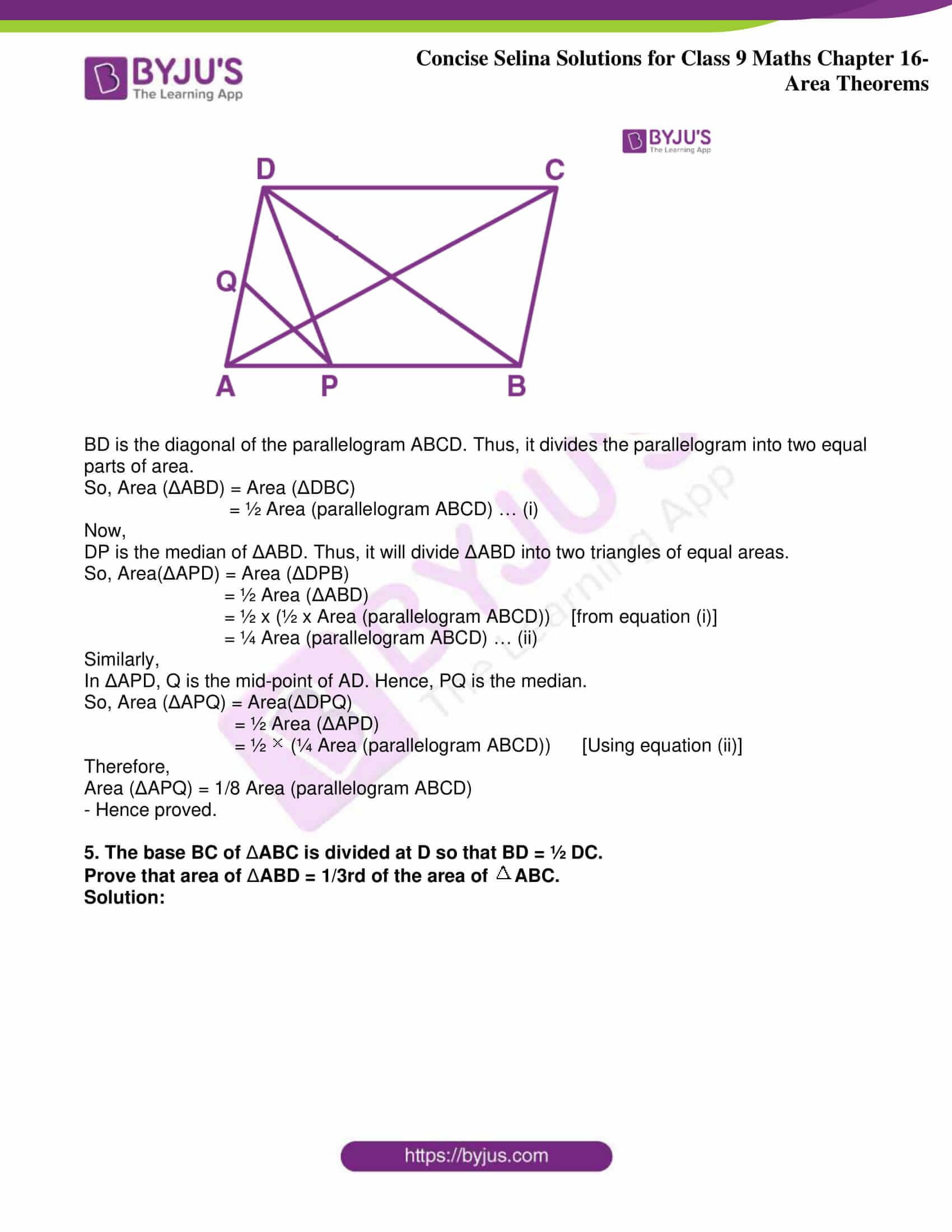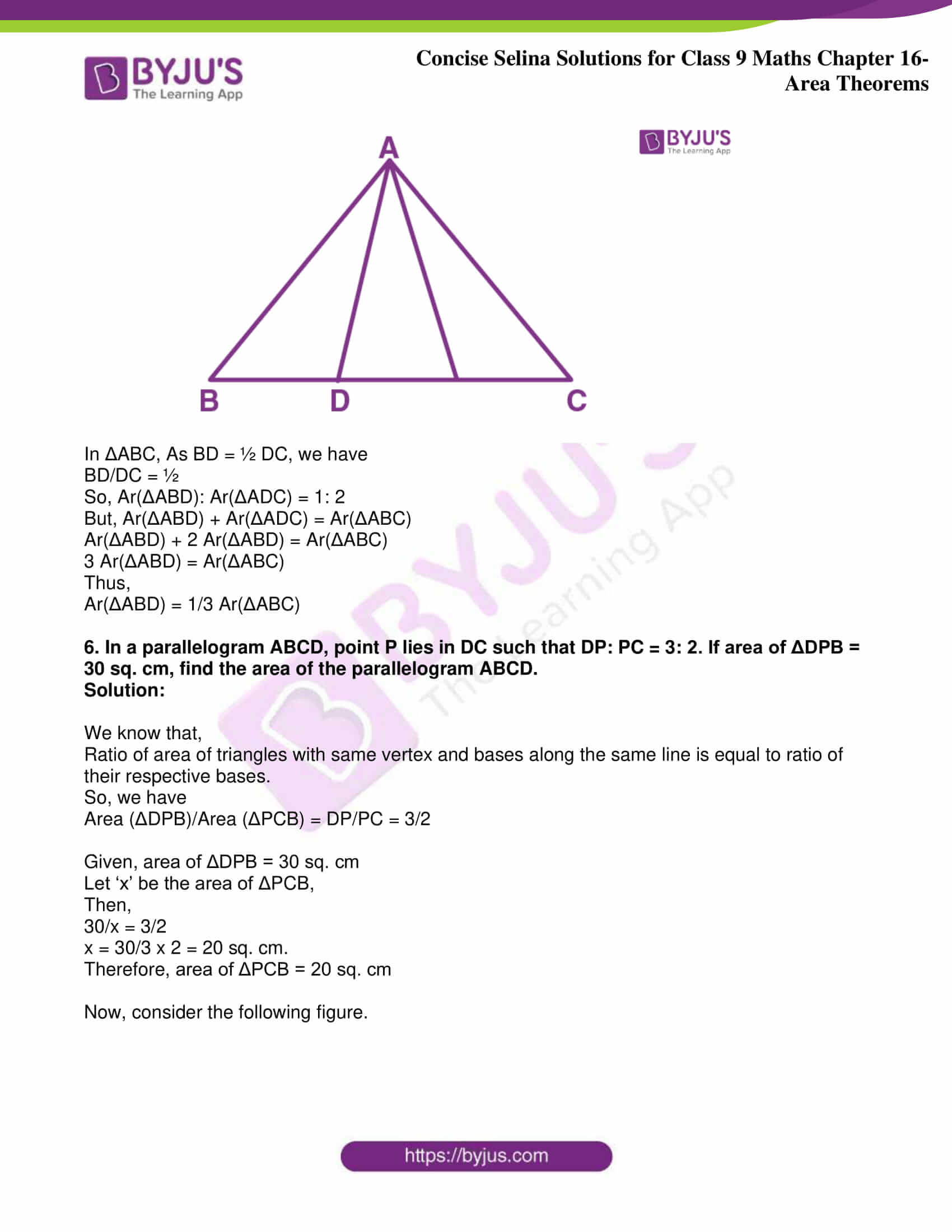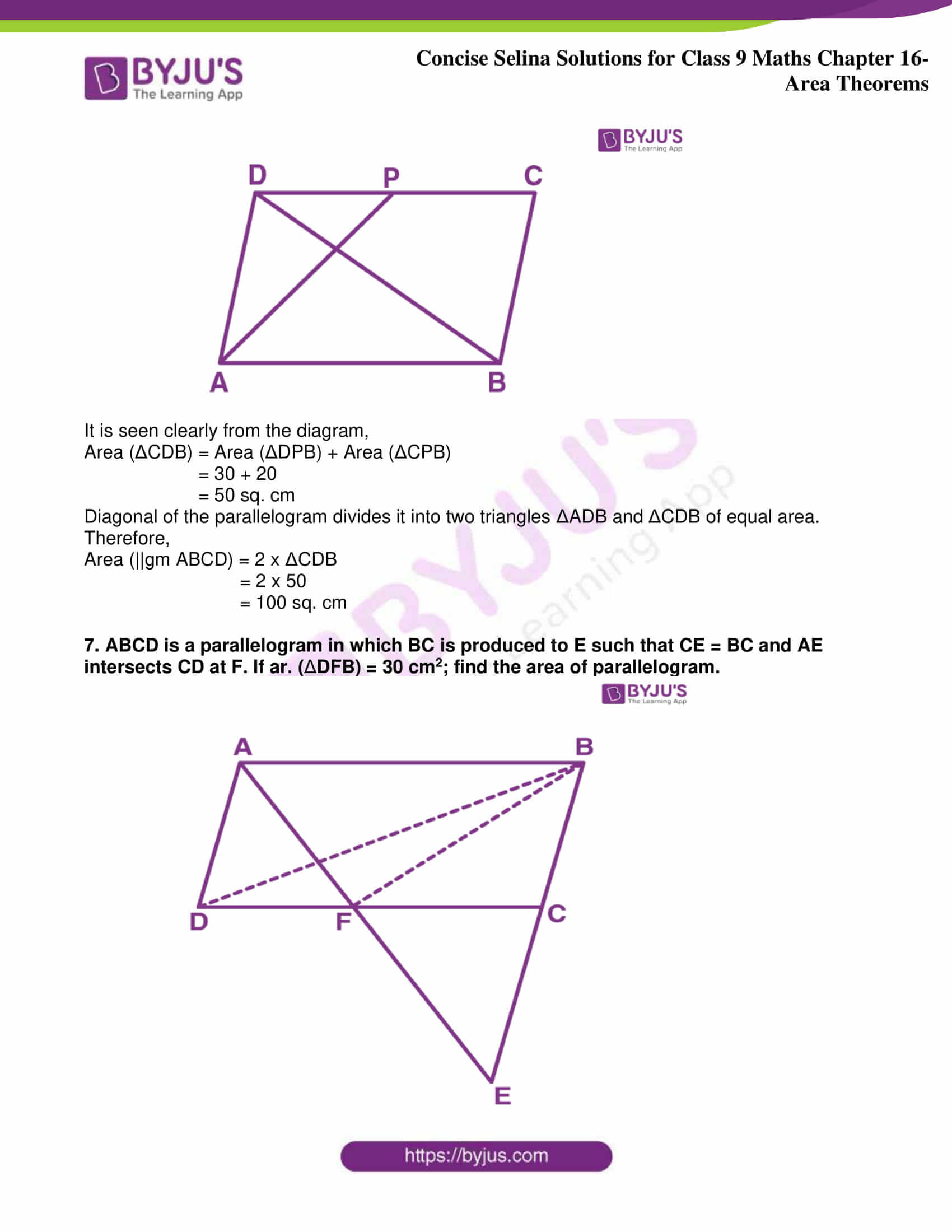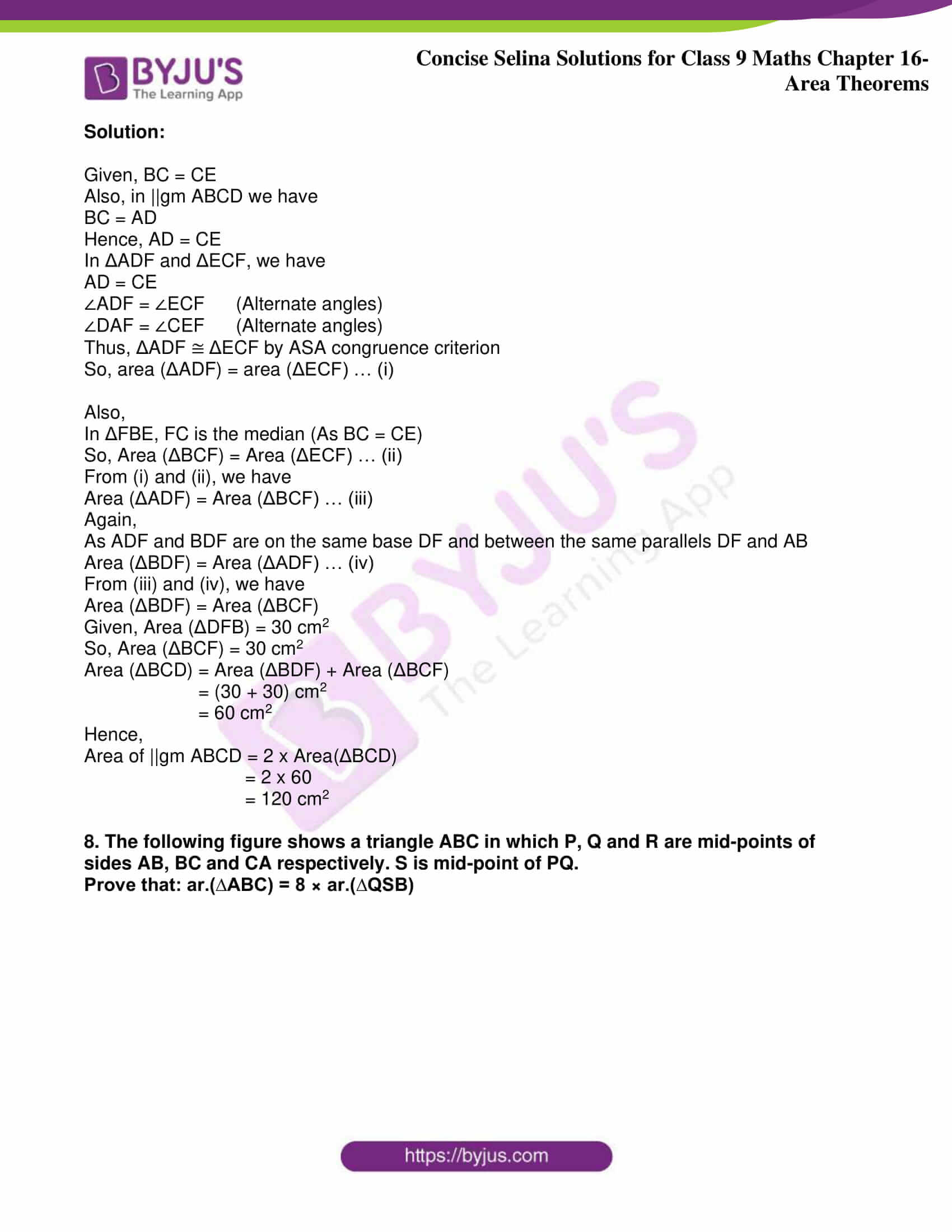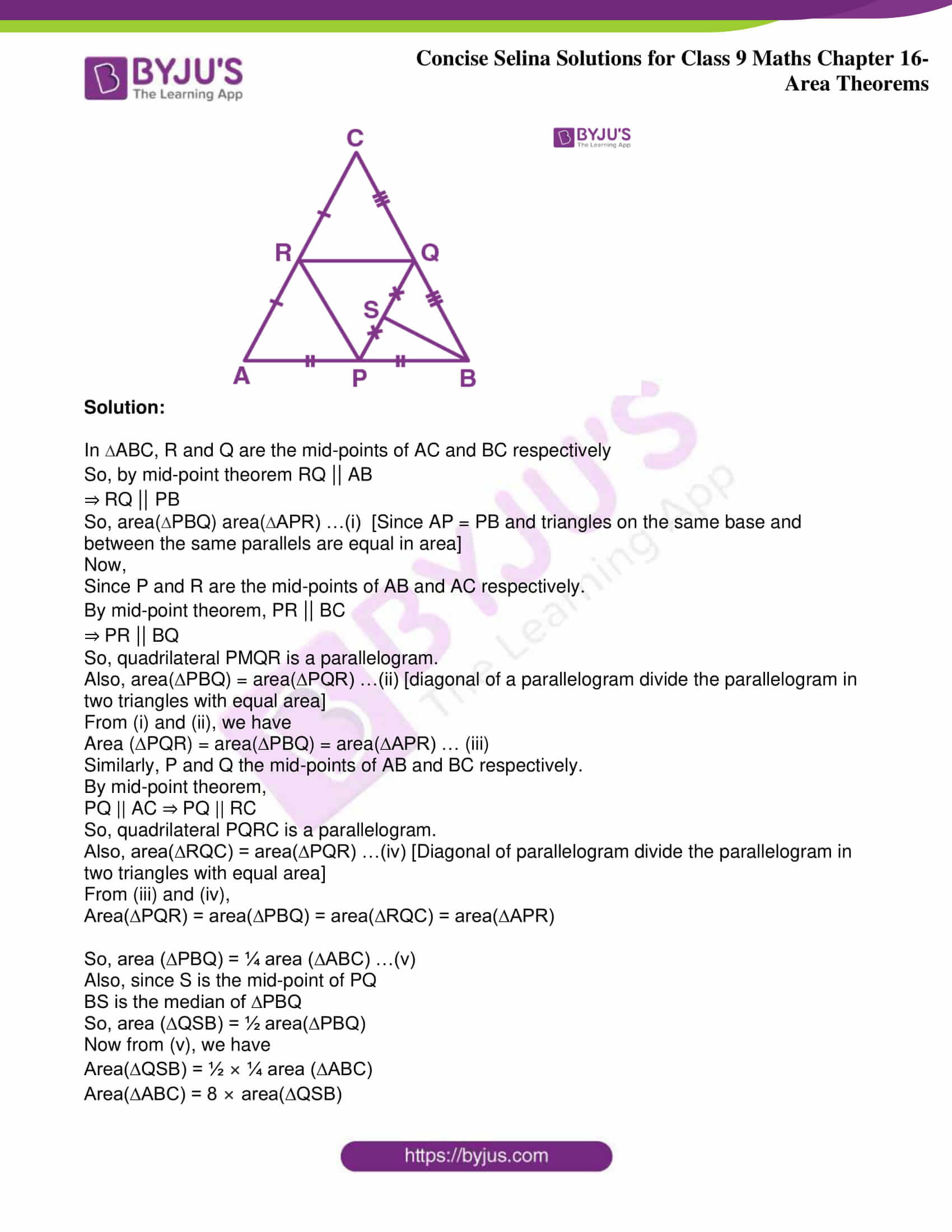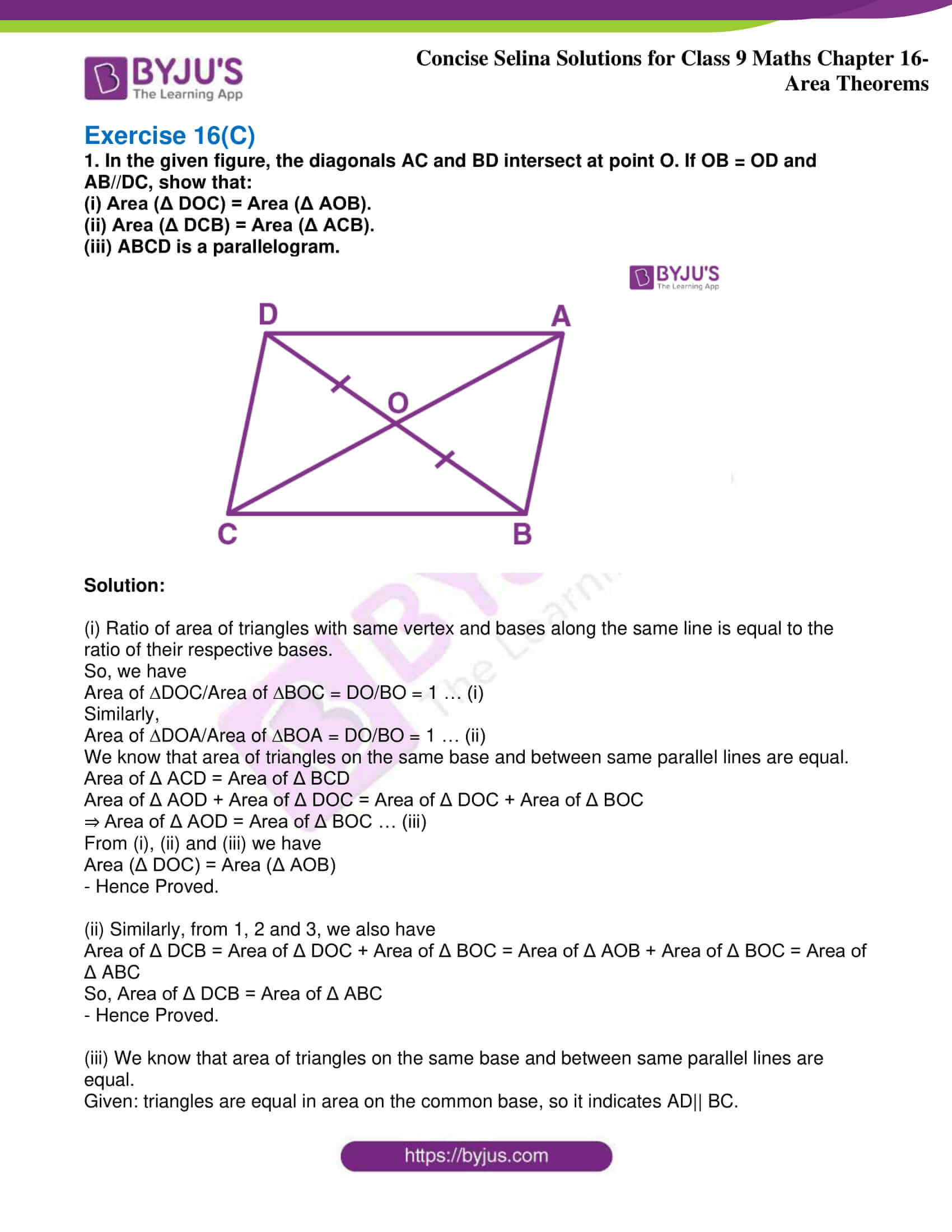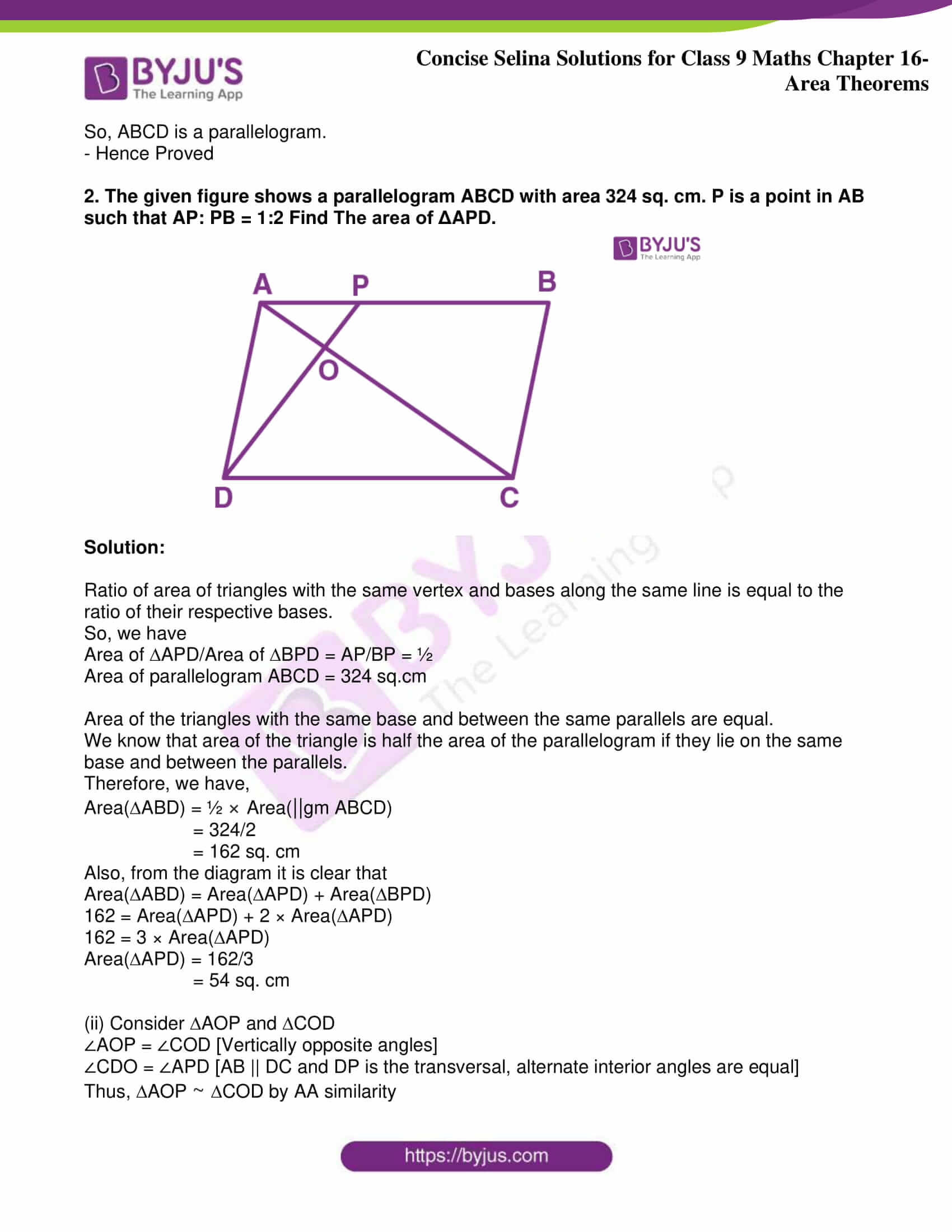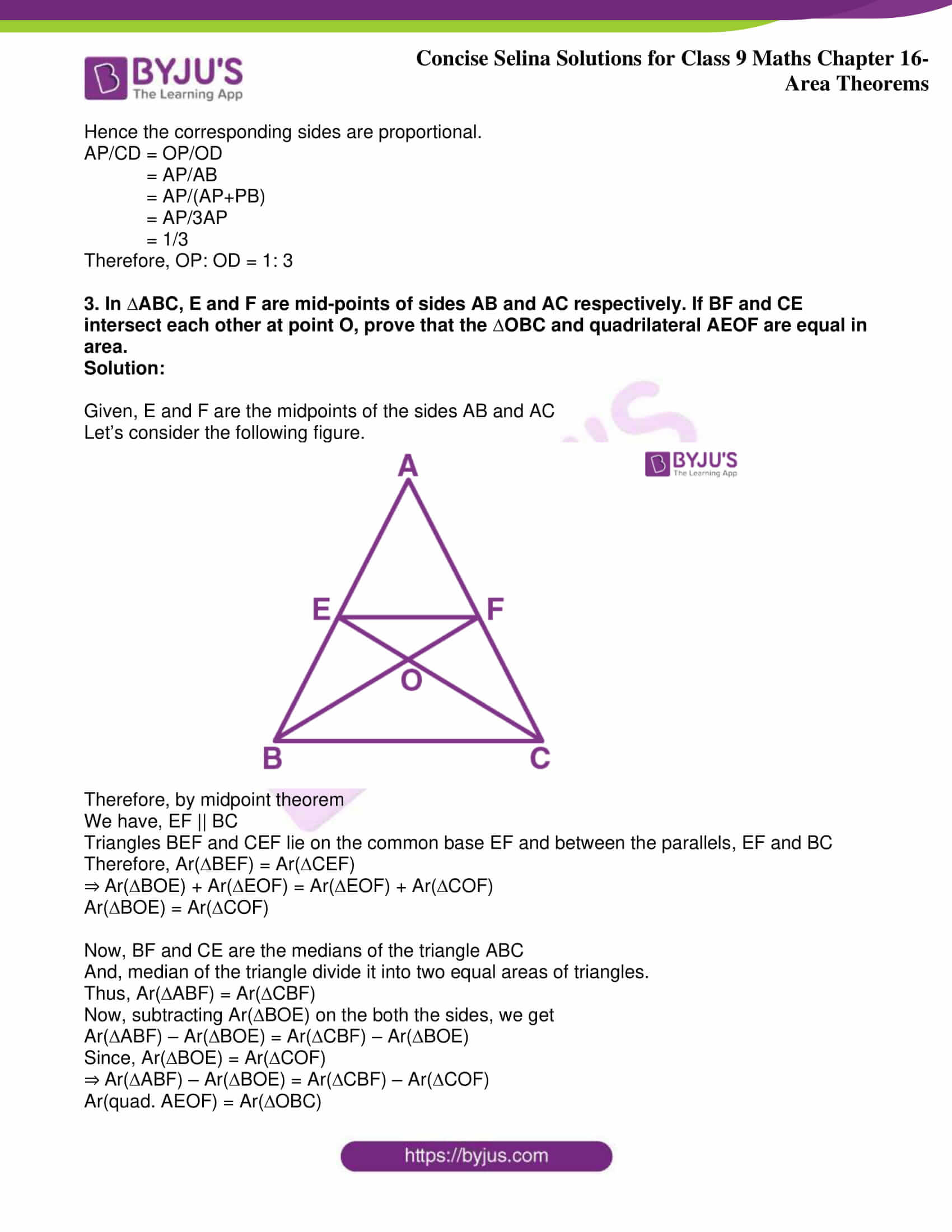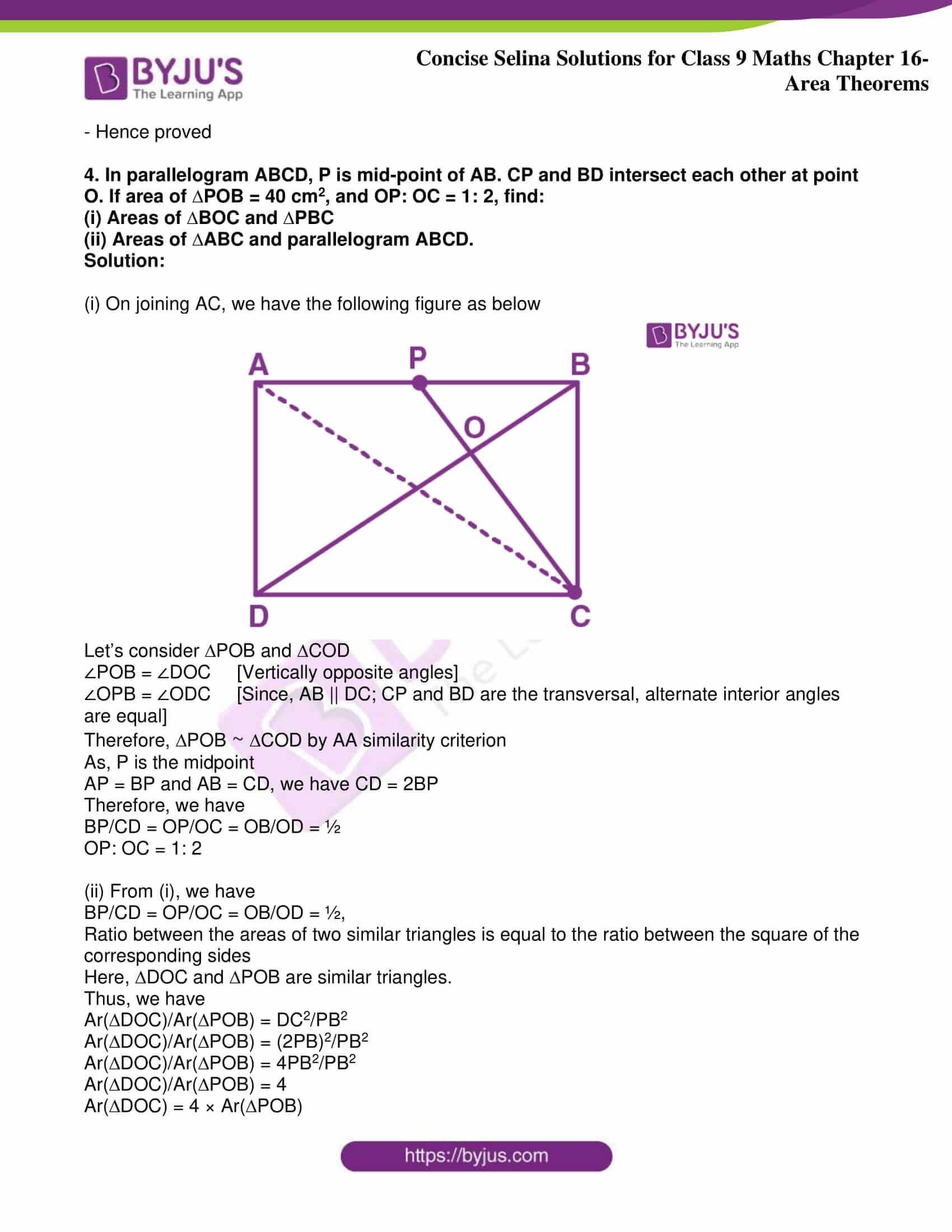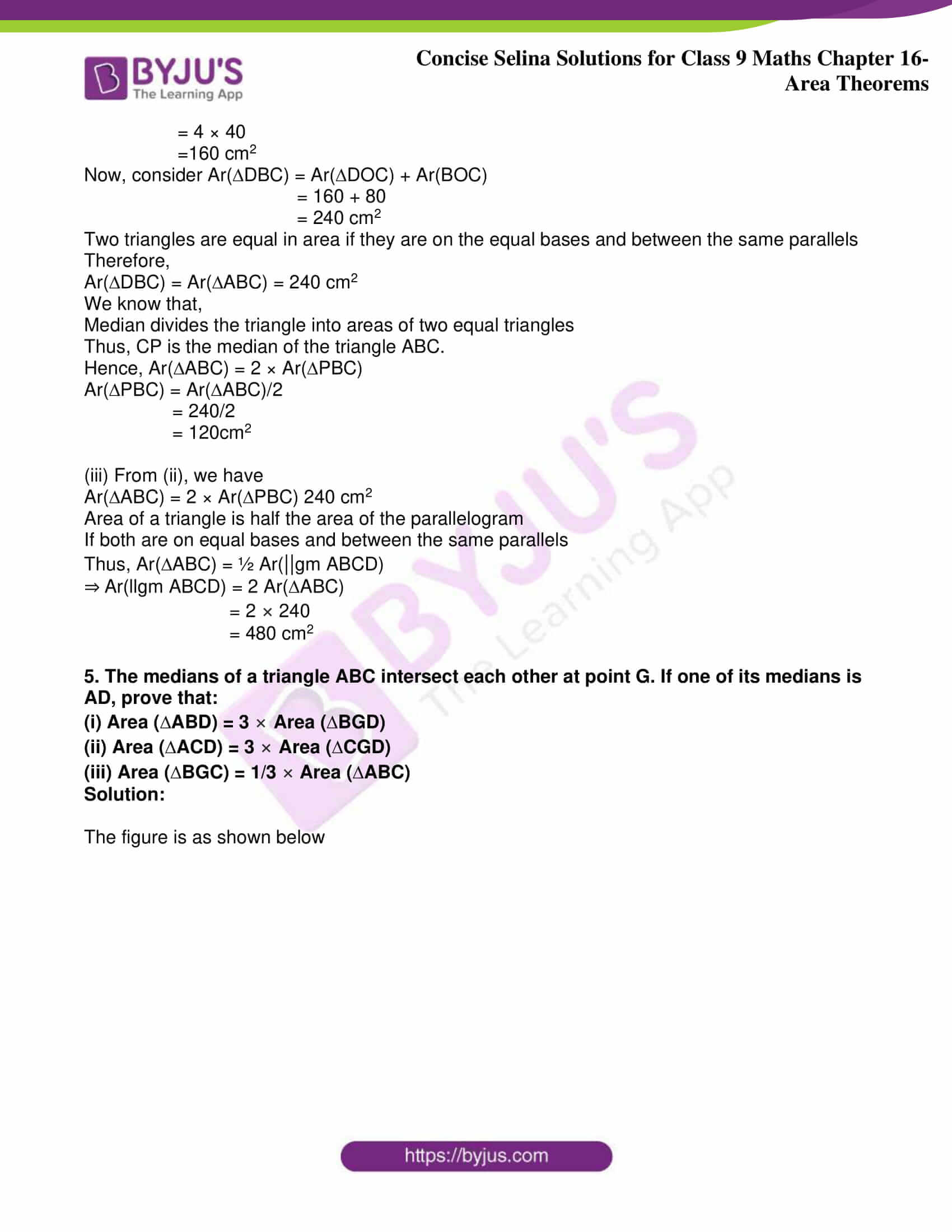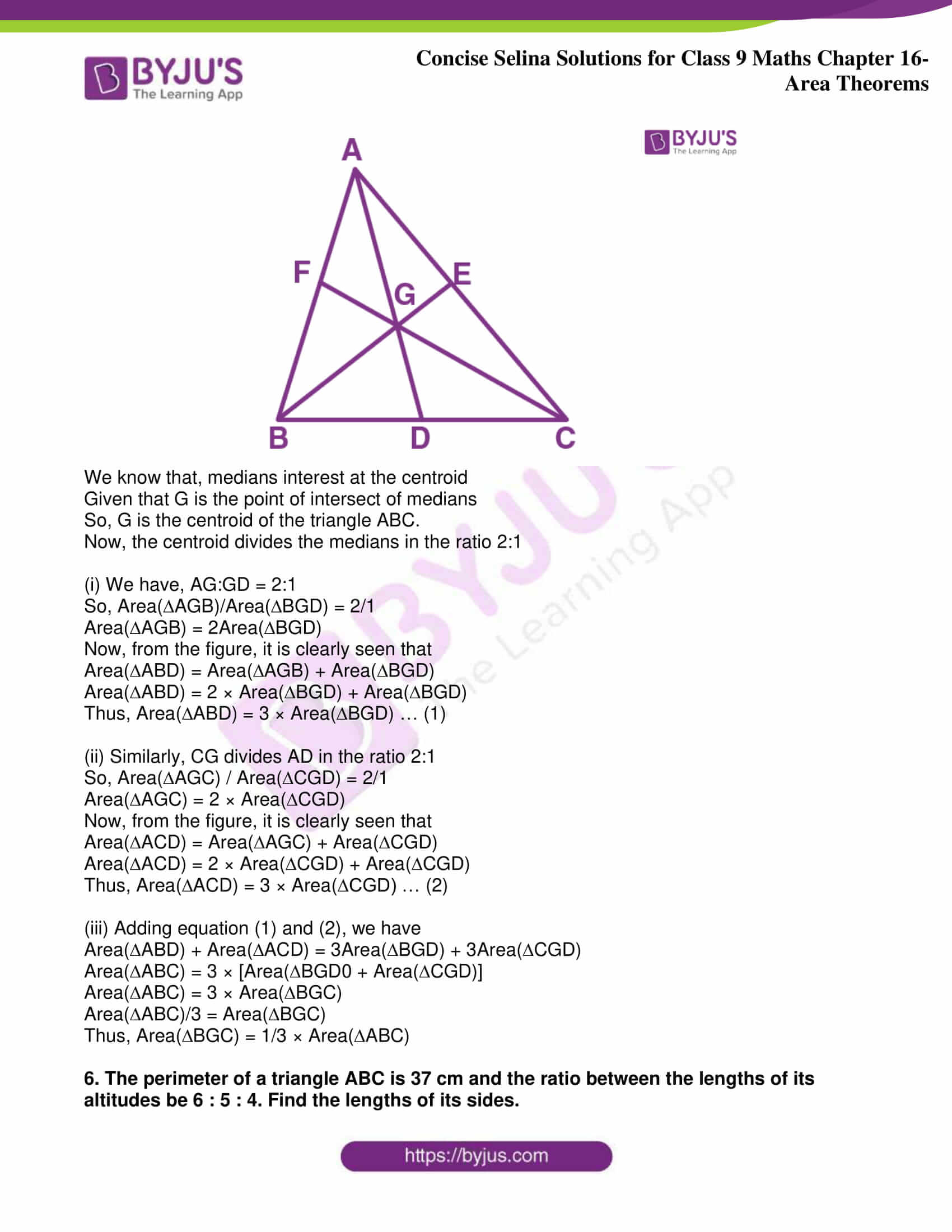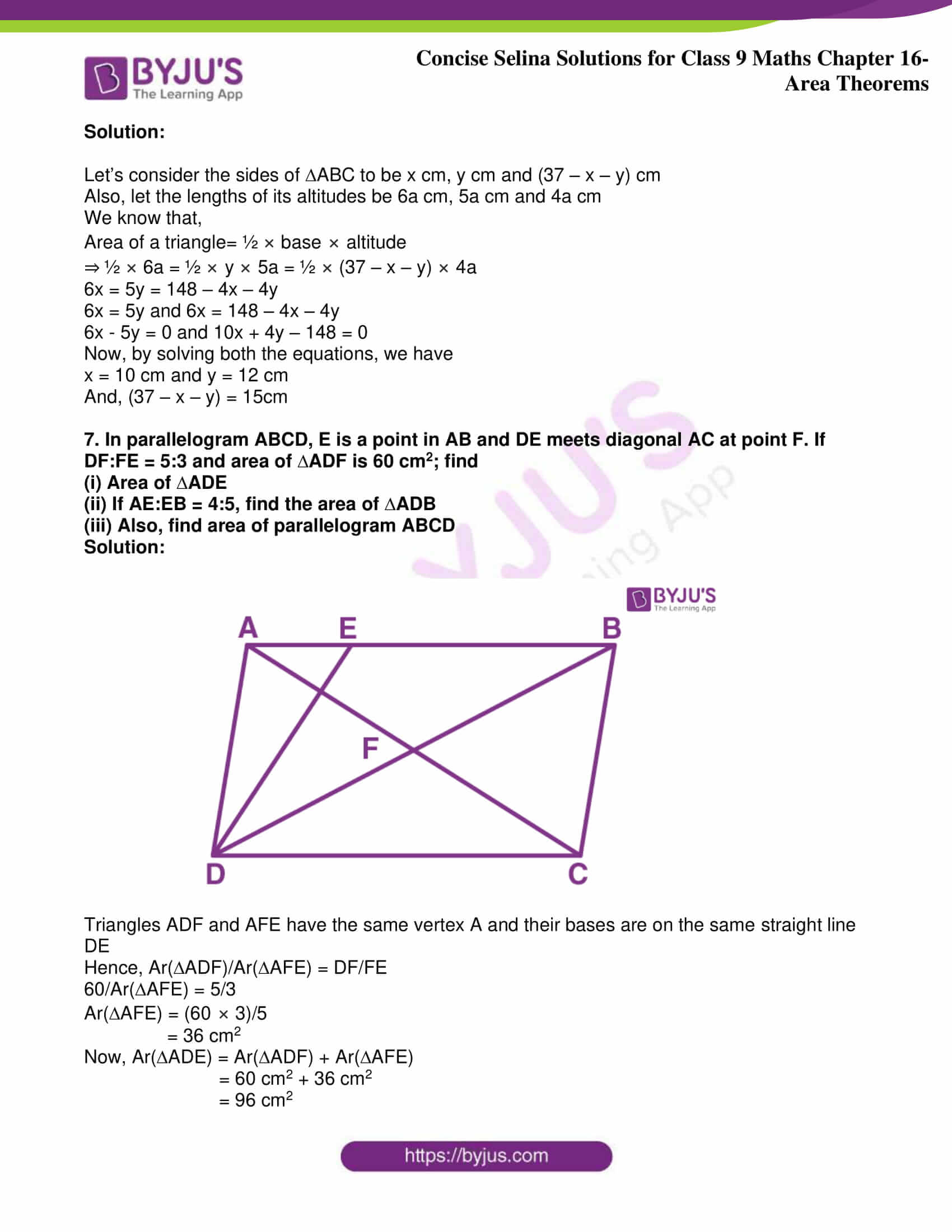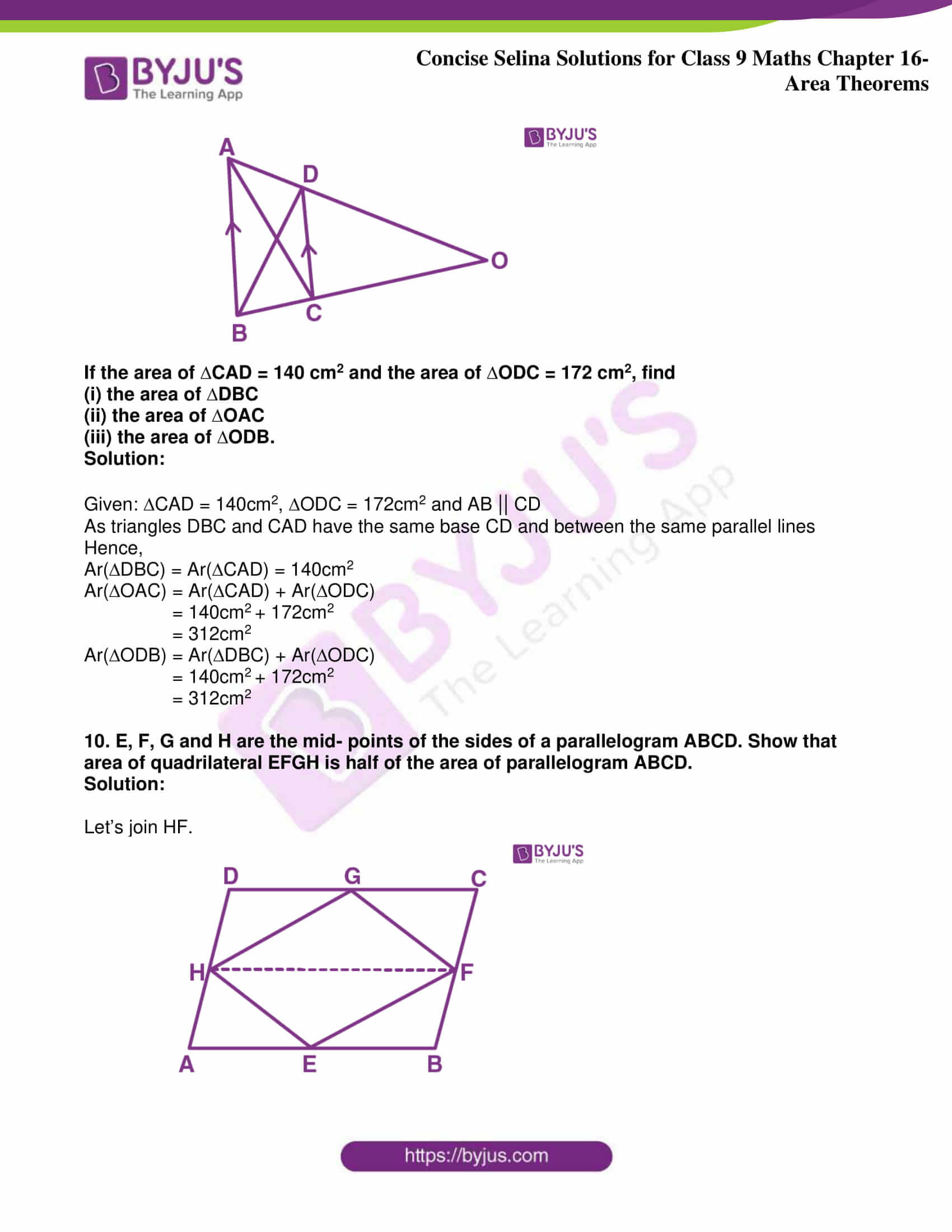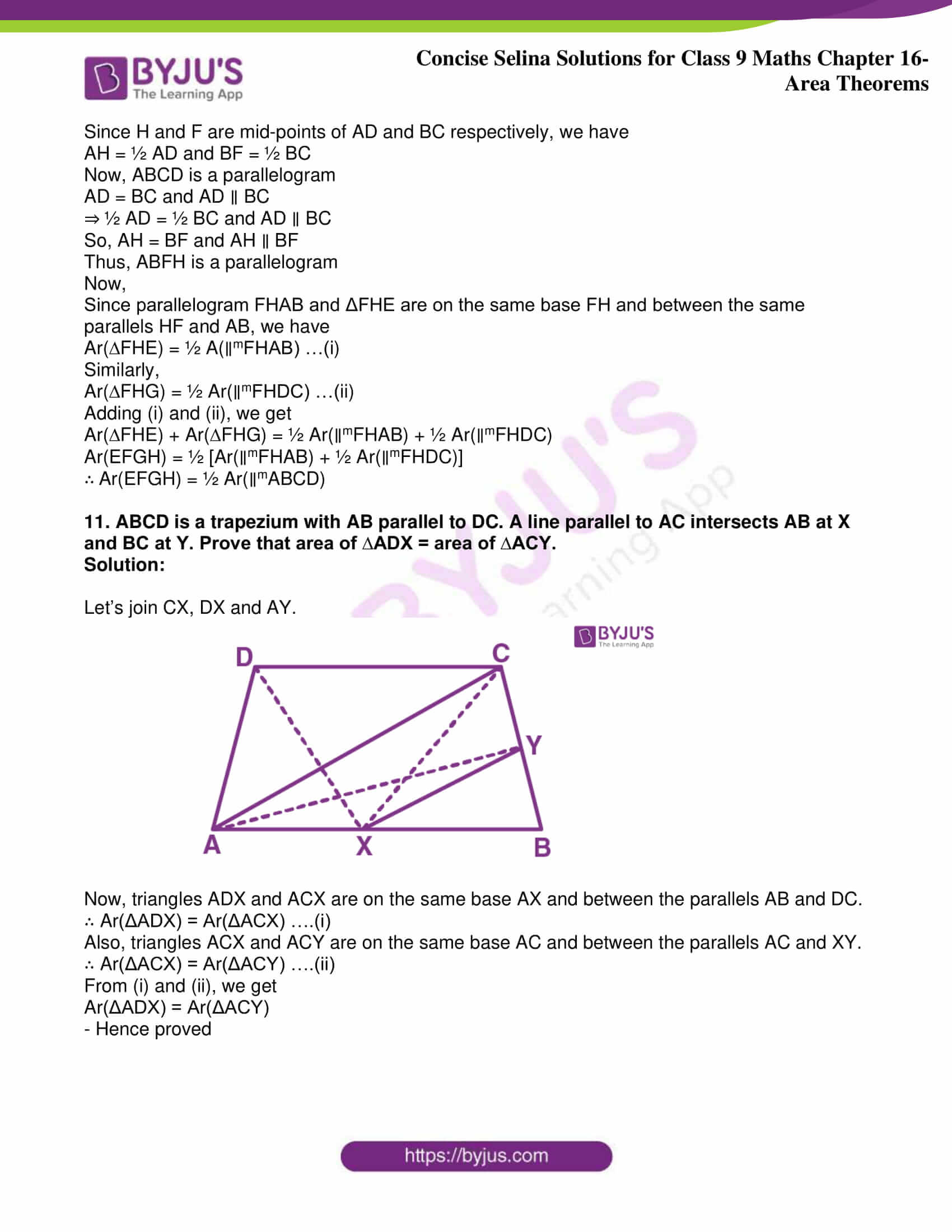Exercise 16(A)

1. In the given figure, if area of triangle ADE is 60 cm2, state, given reason, the area of:

(i) Parallelogram ABED

(ii) Rectangle ABCF

(iii) Triangle ABE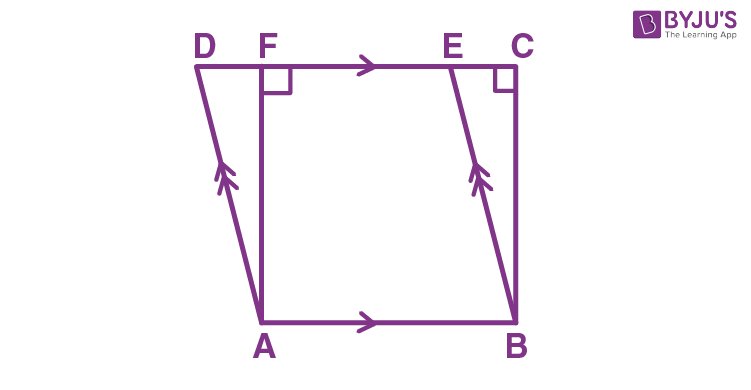Solution:

(i) As ∆ADE and parallelogram ABED are on the same base AB and between the same parallels DE || AB, the area of the ∆ADE will be half the area of parallelogram ABED.

So,

Area of parallelogram ABED = 2 x Area of ∆ADE

= 2 x 60 cm2

= 120 cm2

(ii) Area of parallelogram is equal to the area of rectangle on the same base and of the same altitude i.e., between the same parallels

So,

Area of rectangle ABCF = Area of parallelogram ABED = 120 cm2

(iii) We know that, area of triangles on the same base and between same parallel lines are equal

So,

Area of ∆ABE = Area of ∆ADE = 60 cm2

2. The given figure shows a rectangle ABDC and a parallelogram ABEF; drawn on opposite sides of AB. Prove that:

(i) Quadrilateral CDEF is a parallelogram.

(ii) Area of quad. CDEF = Area of rect. ABDC + Area of || gm. ABEF.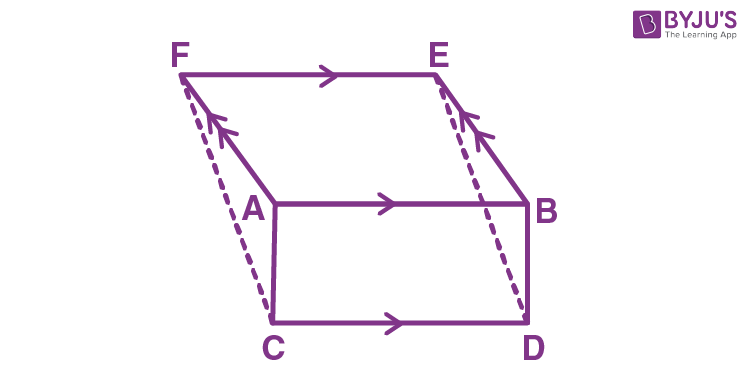Solution:

After drawing the opposite sides of AB, we get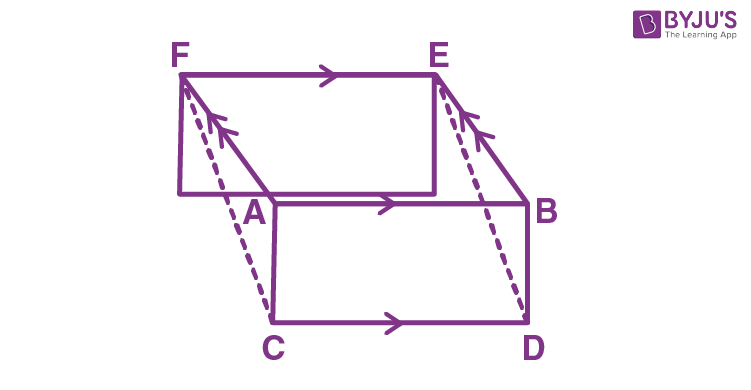It’s seen from the figure that CD|| FE. Therefore, FC must parallel to DE.

Thus, it is proved that the quadrilateral CDEF is a parallelogram.

We know that,

Area of parallelograms on same base and between same parallel lines is always equal. Also, area of parallelogram is equal to the area of rectangle on the same base and of the same altitude i.e., between same parallel lines.

So,

Area of CDEF = Area of ABDC + Area of ABEF

– Hence Proved.

3. In the given figure, diagonals PR and QS of the parallelogram PQRS intersect at point O and LM is parallel to PS. Show that: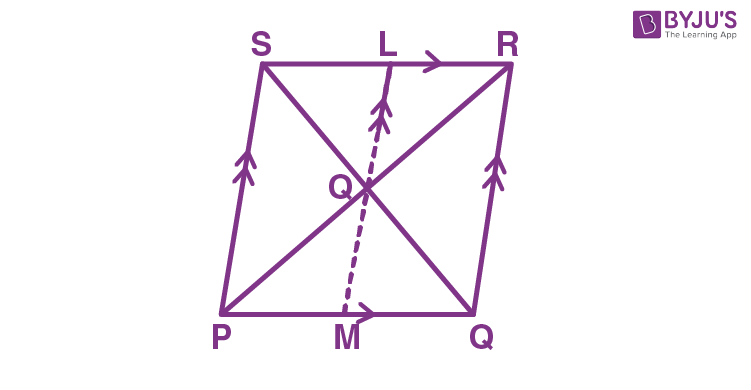(i) 2 Area (∆POS) = Area (||gm PMLS)

(ii) Area (∆POS) + Area (∆QOR) = ½ Area (||gm PQRS)

(iii) Area (∆POS) + Area (∆QOR) = Area (∆POQ) + Area (∆SOR).

Solution:

(i) As POS and parallelogram PMLS are on the same base PS and between the same parallels i.e., SP || LM

Since O is the center of LM and ratio of areas of triangles with same vertex and bases along the same line is equal to ratio of their respective bases

Also, the area of the parallelogram is twice the area of the triangle if they lie on the same base and in between the same parallels

Thus, 2 Area (∆PSO) = Area(PMLS)

(ii) Taking LHS, we have

LM is parallel to PS and PS is parallel to RQ, therefore, LM is parallel to RQ

And, since POS lie on the base PS and in between the parallels PS and LM, we have

Area (POS) = ½ Area (PSLM)

Also, since QOR lie on the base QR and in between the parallels LM and RQ, we have

Area (QOR) = ½ Area (LMQR)

Now,

Area(POS) + Area(QOR) = ½ Area(PSLM) + ½ Area(LMQR)

= ½ [Area(PSLM) + Area(LMQR)]

= ½ Area(PQRS)

(iii) We know that, the diagonals in a parallelogram bisect each other.

So, OS = OQ

In PQS, as OS = OQ

OP is the median of the PQS.

We know that median of a triangle divides it into two triangles of equal area.

Therefore,

Area (POS) = Area (POQ) … (1)

Similarly, as OR is the median of the triangle QRS, we have

Area (QOR) = Area (SOR) … (2)

Now, adding (1) and (2) we get

Area (POS) + area (QOR) = Area (POQ) + Area (SOR)

– Hence Proved.

4. In parallelogram ABCD, P is a point on side AB and Q is a point on side BC.

Prove that:

(i) ∆CPD and ∆AQD are equal in area.

(ii) Area (∆AQD) = Area (∆APD) + Area (∆CPB)

Solution:Given, ABCD is a parallelogram.

P and Q are any points on the sides AB and BC respectively.

Join diagonals AC and BD.

Proof:

(i) As triangles with same base and between same set of parallel lines have equal areas

Area (CPD) = Area (BCD) …… (1)

Again, diagonals of the parallelogram bisect area in two equal parts

Area (BCD) = ½ area of parallelogram ABCD …… (2)

From (1) and (2)

Area (CPD) = ½ Area (ABCD) …… (3)

Similarly,

Area (AQD) = Area (ABD) = ½ Area (ABCD) …… (4)

From (3) and (4), we get

Area (CPD) = Area (AQD)

– Hence proved.

(ii) We know that, area of triangles on the same base and between same parallel lines are equal

So,

Area of AQD = Area of ACD = Area of PDC = Area of BDC = Area of ABC = Area of APD + Area of BPC

– Hence Proved

5. In the given figure, M and N are the mid-points of the sides DC and AB respectively of the parallelogram ABCD.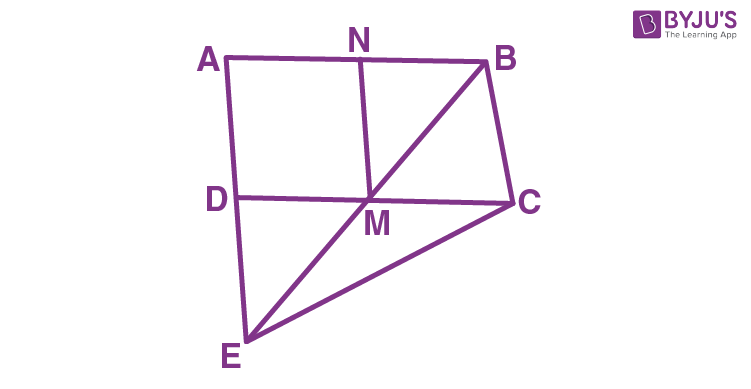If the area of parallelogram ABCD is 48 cm2

(i) State the area of the triangle BEC.

(ii) Name the parallelogram which is equal in area to the triangle BEC.

Solution:

(i) As BEC and parallelogram ABCD are on the same base BC and between the same parallels i.e., BC || AD.

Area (BEC) = ½ x Area (ABCD) = ½ x 48 = 24 cm2

(ii) Area (ANMD) = Area (BNMC)

= ½ x Area (ABCD)

= ½ x 2 x Area (BEC)

= Area (BEC)

Therefore, the parallelograms ANMD and NBCM have areas equal to BEC.

6. In the following figure, CE is drawn parallel to diagonals DB of the quadrilateral ABCD which meets AB produced at point E.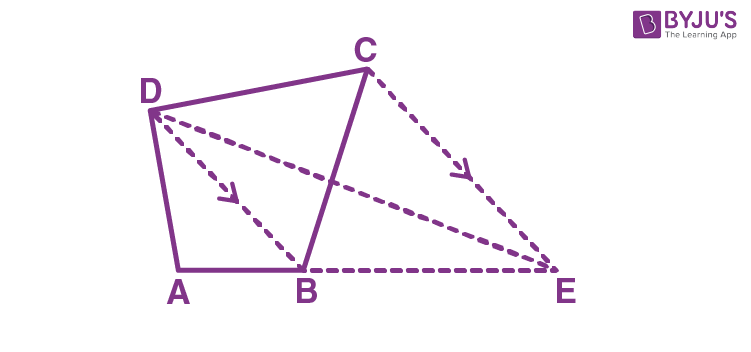Solution:

As, DCB and DEB are on the same base DB and between the same parallels i.e., DB || CE, We have,

Area (DCB) = Area (DEB)

– Hence proved

7. ABCD is a parallelogram a line through A cuts DC at point P and BC produced at Q. Prove that triangle BCP is equal in area to triangle DPQ.Solution:

Its seen that, APB and parallelogram ABCD are on the same base AB and between the same parallel lines AB and CD.

So,

Ar(APB) = ½ Ar(||gm ABCD) … (i)

Now,

ADQ and parallelogram ABCD are on the same base AD and between the same parallel lines AD and BQ.

So,

Ar(ADQ) = ½ Ar(||gm ABCD) … (ii)

On adding equation (i) and (ii), we get

Ar(APB) + Ar(
ADQ) = ½ Ar(||gm ABCD) + ½ Ar(||gm ABCD) = Ar(||gm ABCD)

Ar(BPQ) = Ar(DCQ)

Subtracting Ar(PCQ) from both the sides, we get

Ar(BPQ) – Ar(PCQ) = Ar(DCQ) – Ar(PCQ)

Ar(BCP) = Ar(DPQ)

– Hence proved.

8. The given figure shows a pentagon ABCDE. EG drawn parallel to DA meets BA produced at G and CF draw parallel to DB meets AB produced at F.

Prove that the area of pentagon ABCDE is equal to the area of triangle GDF.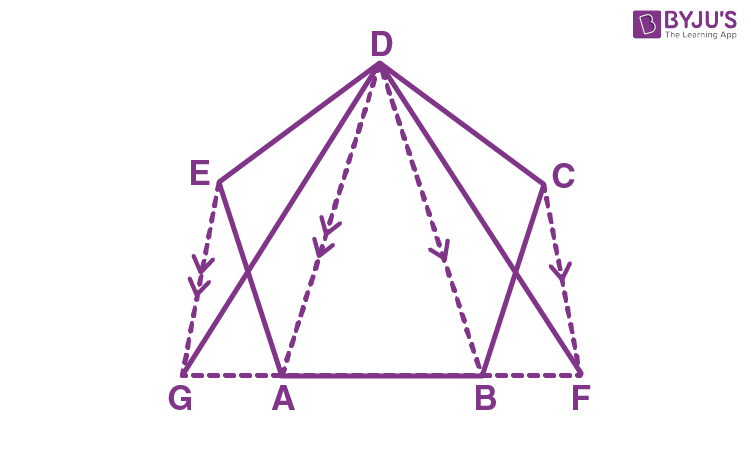Solution: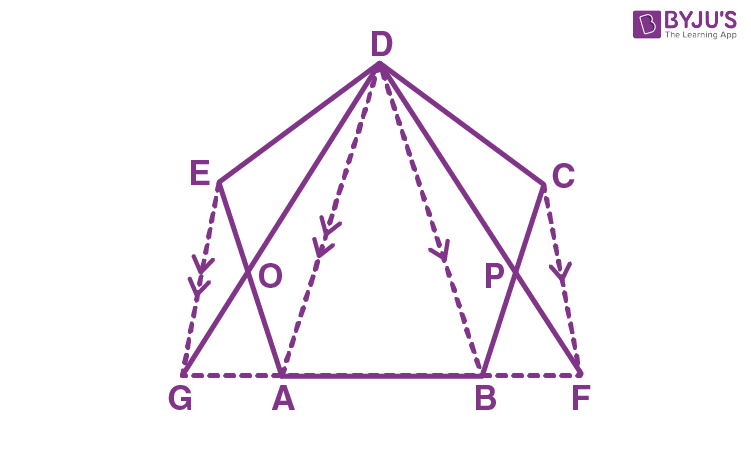It’s seen that triangles EDG and EGA are on the same base EG and between the same parallel lines EG and DA, so

Ar(EDG) = Ar(EGA)

On subtracting EOG from both sides, we have

Ar(EDG) – Ar(EOG) = Ar(EGA) – Ar(EOG)

Ar(EOD) = Ar(GOA) … (i)

Similarly,

Ar(DPC) = Ar(BPF) … (ii)

Now,

Ar(GDF) = Ar(GOA) + Ar(BPF) + Ar(pen. ABPDO)

= Ar(EOD) + Ar(DPC) + Ar(pen. ABPDO)

= Ar(pen. ABCDE)

– Hence proved

9. In the given figure, AP is parallel to BC, BP is parallel to CQ. Prove that the area of triangles ABC and BQP are equal.Solution:

On joining PC, we see thatABC and BPC are on the same base BC and between the same parallel lines AP and BC.

Ar(ABC) = Ar(BPC) … (i)

And, BPC and BQP are on the same base BP and between the same parallel lines BP and CQ.

Ar(BPC) = Ar(BQP) … (ii)

From (i) and (ii), we get

Ar(ABC) = Ar(BQP)

– Hence proved.

10. In the figure given alongside, squares ABDE and AFGC are drawn on the side AB and the hypotenuse AC of the right triangle ABC.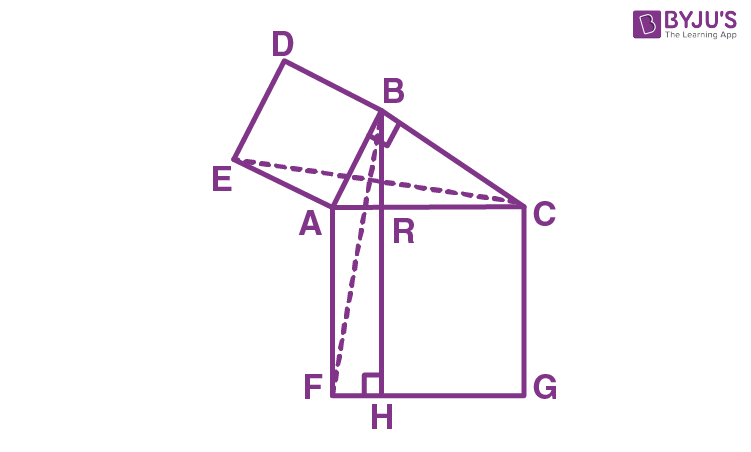If BH is perpendicular to FG prove that:

(i) ∆EAC ∆BAF.

(ii) Area of the square ABDE = Area of the rectangle ARHF.

Solution:

(i) From figure,

∠EAC = ∠EAB + ∠BAC

= 90o + ∠BAC … (i)

∠BAF = ∠FAC + ∠BAC

= 90o + ∠BAC … (ii)

From (i) and (ii), we get

∠EAC = ∠BAF

In EAC and BAF, we have,

EA = AB

∠EAC = ∠BAF (proved above)

AC = AF

Therefore, EAC ≅ BAF (SAS axiom of congruency)

(ii) As ABC is a right triangle, we have

AC2 = AB2 + BC2 [Using Pythagoras theorem in ∆ABC]

AB2 = AC2 – BC2

AB2 = (AB2 + BC2) – [BR2 + RC2] [Since AC2 = AR2 + RC2and using Pythagoras Theorem in ∆BRC]

AB2 = AR2+ 2AR × RC + RC2– (BR2+ RC2) [Using the identity]

AB2 = AR2+ 2AR × RC + RC2– (AB2– AR2+ RC2) [Using Pythagoras Theorem in ∆ABR ]

2AB2 = 2AR2+ 2AR × RC

AB2 = AR (AR + RC)

AB2 = AR × AF

∴ Area (ABDE) = Area (rectangle ARHF)

11. In the following figure, DE is parallel to BC. Show that:

(ii) Area (∆BOD) = Area (∆COE).Solution:

(i) In ABC, D is midpoint of AB and E is the midpoint of AC

And, DE is parallel to BC

So, by mid-point theorem, we have

Ar(ADC) = Ar(BDC) = ½ Ar(ABC) … (i)

Again,

Ar(AEB) = Ar(BEC) = ½ Ar(ABC) … (ii)

From equations (i) and (ii), we have

– Hence Proved

(ii) We know that, area of triangles on the same base and between same parallel lines are equal

So, Area(DBC) = Area( BCE)

Area(DOB) + Area(BOC) = Area(BOC) + Area(COE)

Thus, Area(DOB) = Area(COE) [On subtracting Area(BOC) on both sides]

12. ABCD and BCFE are parallelograms. If area of triangle EBC = 480 cm2; AB = 30 cm and BC = 40 cm; Calculate:

(i) Area of parallelogram ABCD

(ii) Area of the parallelogram BCFE

(iii) Length of altitude from A on CD

(iv) Area of triangle ECF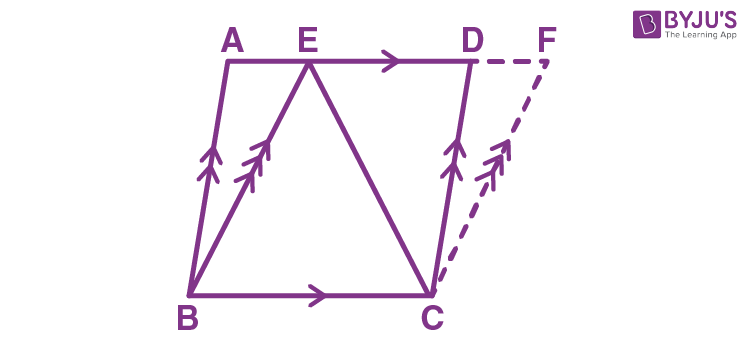Solution:

(i) As EBC and parallelogram ABCD are on the same base BC and between the same parallels i.e., BC||AD.

So, Area(EBC) = ½ Area(||gm ABCD)

Area(||gm ABCD) = 2 x Ar(EBC)

= 2 x 480 cm2

= 960 cm2

(ii) We know that, parallelograms on same base and between same parallels are equal in area

So, Area of BCFE = Area of ABCD = 960 cm2

(iii) Area of ACD = 960/2 = 480

= (1/2) x 30 x Altitude

Thus,

Altitude = 480/15

= 32 cm

(iv) We know that, the area of a triangle is half that of a parallelogram on the same base and between the same parallels

Therefore,

Area(ECF) = ½ Area(||gm CBEF)

Similarly,

Area(BCE) = ½ Area(||gm CBEF)

Thus,

Area(ECF) = Area(BCE) = 960/2 = 480 cm2

13. In the given figure, D is mid-point of side AB of ∆ABC and BDEC is a parallelogram.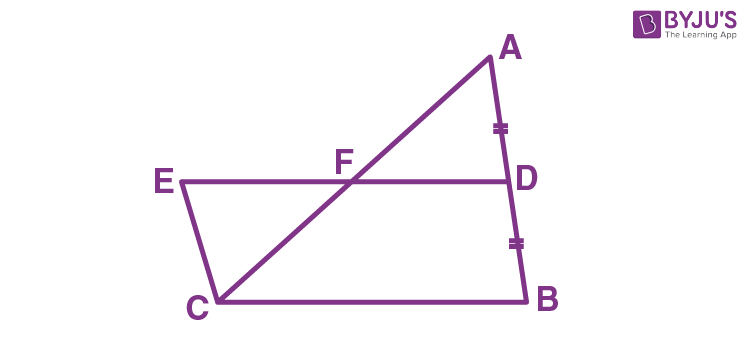Prove that: Area of ∆ABC = Area of ||gm BDEC.

Solution:

Here, AD = DB and EC = DB (Given)

It’s seen that EFC = AFD (Vertically opposite angles)

And, as ED and CB are parallel lines with AC cutting these lines, we have

∠ECF = ∠FAD (Alternate interior angles)

From the above conditions, we have

EFC ≅ AFD by AAS Congruency criterion

So, Area (EFC) = Area (AFD)

Area of || gm BDEC = Area of ABC

– Hence proved

14. In the following, AC || PS || QR and PQ || DB || SR.

Prove that:Solution:

In parallelogram PQRS, AC || PS || QR and PQ || DB || SR

Similarly, AQRC and APSC are also parallelograms.

As, ABC and parallelogram AQRC are on the same base AC and between the same parallels, we have

Ar(ABC) = ½ Ar(AQRC)……(i)

Similarly,

On adding (i) and (ii), we get

Ar(ABC) + Ar(ADC) = ½ Ar(AQRC) + ½ Ar(APSC)

Therefore,

15. ABCD is trapezium with AB || DC. A line parallel to AC intersects AB at point M and BC at point N. Prove that: area of ΔADM = area of ΔACN.

Solution:

Given: ABCD is a trapezium

AB || CD, MN || AC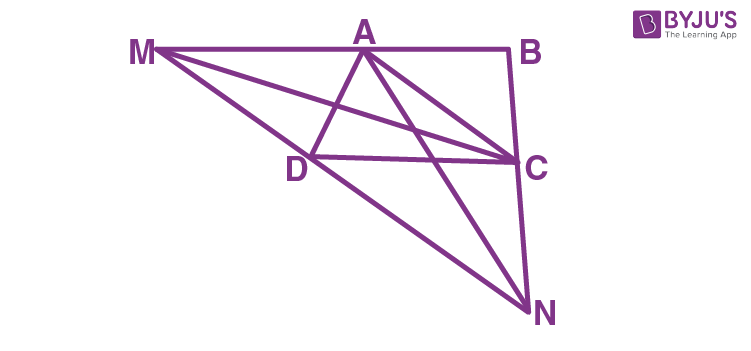Let’s join C and M

We know that, area of triangles on the same base and between same parallel lines are equal.

So, Area of ΔAMD = Area of ΔAMC … (i)

Similarly, considering quad. AMNC where MN || AC, we have

ΔACM and ΔACN are on the same base and between the same parallel lines

Thus, their areas should be equal.

i.e., Area of ΔACM = Area of ΔCAN … (ii)

From equations (i) and (ii), we get

Area of ΔADM = Area of ΔCAN

– Hence Proved.

16. In the given figure, AD || BE || CF. Prove that area (ΔAEC) = area (ΔDBF)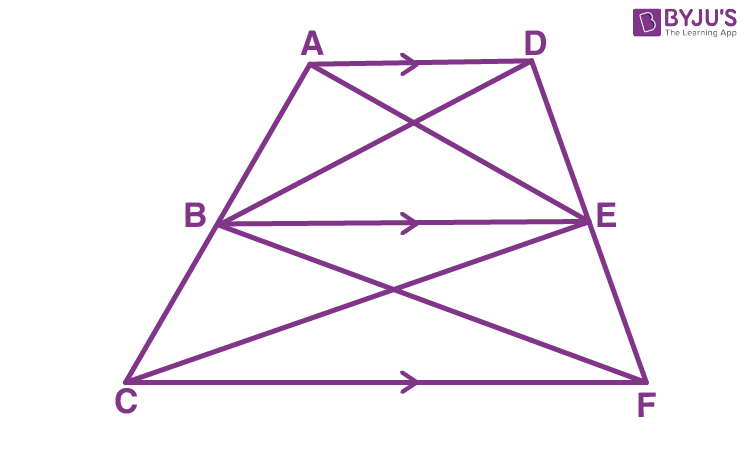Solution:

We know that,

Area of triangles on the same base and between same parallel lines are equal.

With common base, BE and between AD and BE parallel lines, we have

Area of ΔABE = Area of ΔBDE … (i)

Similarly, in BEFC quadrilateral and BE||CF

With common base BC and between BE and CF parallel lines, we have

Area of ΔBEC = Area of ΔBEF … (ii)

On adding equations (i) and (ii), we get

Area of ΔABE + Area of ΔBEC = Area of ΔBEF + Area of ΔBDE

Thus,

Area of ΔAEC = Area of ΔDBF

– Hence Proved

17. In the given figure, ABCD is a parallelogram; BC is produced to point X. Prove that: area (Δ ABX) = area (quad. ACXD).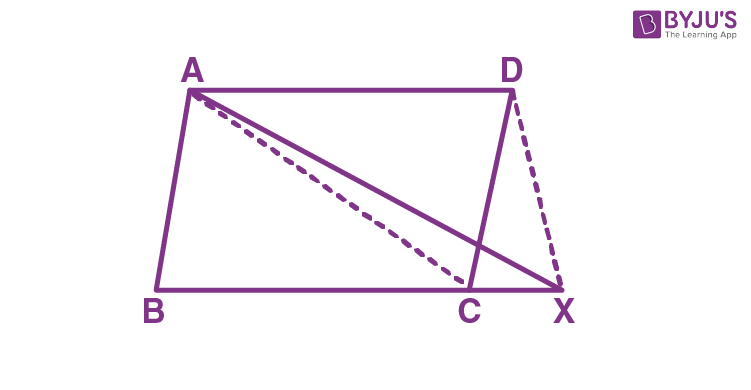Solution:

Given: ABCD is a parallelogram.

We know that,

Area of ΔABC = Area of ΔACD (Diagonal divides a ||gm into 2 triangles of equal area)

Now, consider ΔABX

Area of ΔABX = Area of ΔABC + Area of ΔACX

We also know that, area of triangles on the same base and between same parallel lines are equal.

So, Area of ΔACX = Area of ΔCXD

From above equations, we have

Area of ΔABX = Area of ΔABC + Area of ΔACX

= Area of ΔACD+ Area of ΔCXD

– Hence Proved

18. The given figure shows parallelograms ABCD and APQR. Show that these parallelograms are equal in area.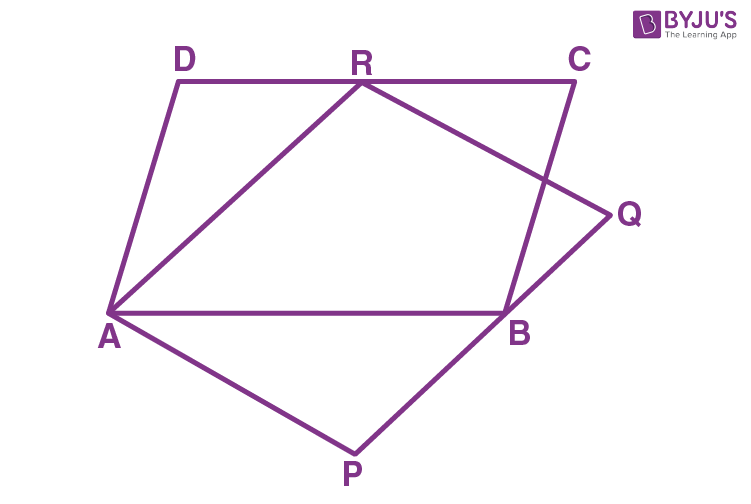Solution:

Let’s join B and R and also P and R.

We know that, the area of the parallelogram is equal to twice the area of the triangle, if the triangle and the parallelogram are on the same base and between the parallels

Taking ABCD parallelogram:

As ||gm ABCD and ΔABR lie on AB and between the parallels AB and DC, we have

Area (||gm ABCD) = 2 x Area (ΔABR) … (i)

Also, the area of triangles with same base and between the same parallel lines are equal.

As the triangles ABR and APR lie on the same base AR and between the parallels AR and QP, we have,

Area (ΔABR) = Area (ΔAPR) … (ii)

From equations (i) and (ii), we have

Area (||gm ABCD) = 2 x Area (ΔAPR) … (iii)

Also, its seen that APR and ||gm ARQP lie on the same base AR and between the same parallels AR and QP

So, Area (ΔAPR) = ½ Area (||gm ARQP) … (iv)

Using (iv) in (iii), we get

Area (||gm ABCD) = 2 x ½ x Area (||gm ARQP)

Area (||gm ABCD) = Area (||gm ARQP)

– Hence proved

Exercise 16(B)

1. Show that:

(i) A diagonal divides a parallelogram into two triangles of equal area.

(ii) The ratio of the areas of two triangles of the same height is equal to the ratio of their bases.

(iii) The ratio of the areas of two triangles on the same base is equal to the ratio of their heights.

Solution:

(i) Let ABCD be a parallelogram (Given)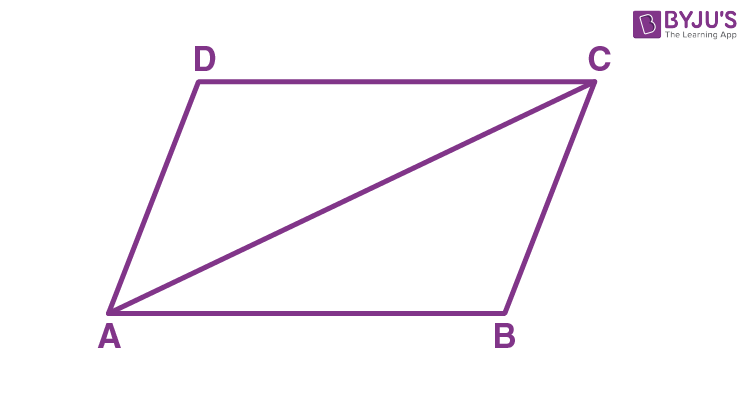Considering the triangles ABC and ADC, we have

AB = CD (opposite sides of ||m)

AD = BC (opposite sides of ||gm)

Thus, ΔABC ≅ ΔADC by SSS congruence criterion

Area of congruent triangles are equal.

Therefore, Area (ΔABC) = Area (ΔADC)

(ii) Consider the following figure: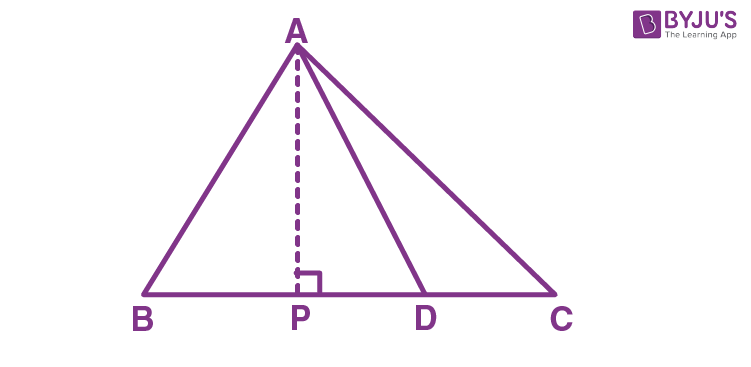Here AP ⊥ BC,

We have,

Ar.(ΔABD) = ½ BD x AP

And, Ar.(ΔADC) = ½ DC x AP

Thus,

Area(ΔABD)/Area(ΔADC) = (½ × BD × AP)/ (½ × DC × AP)

= BD/DC

– Hence proved

(iii) Consider the following figure: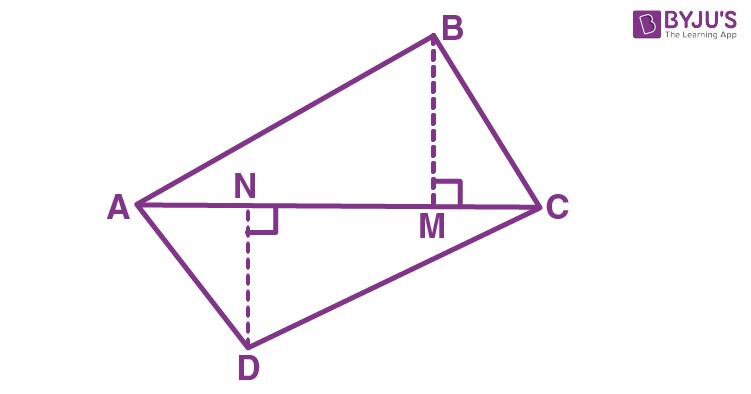Here,

Ar.(ΔABC) = ½ BM x AC

And, Ar.(ΔADC) = ½ DN x AC

Thus,

Area(ΔABC)/Area(ΔADC) = (½ × BM × AC)/ (½ × DN × AC)

= BM/DN

– Hence proved

2. In the given figure; AD is median of ΔABC and E is any point on median AD. Prove that Area (ΔABE) = Area (ΔACE).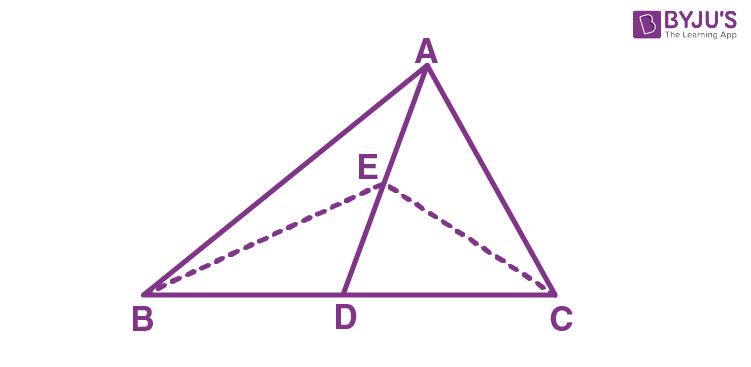Solution:

As AD is the median of ΔABC, it will divide ΔABC into two triangles of equal areas.

So, Area (ΔABD) = Area (ΔACD) … (i)

Also, since ED is the median of ΔEBC

So, Area (ΔEBD) = Area (ΔECD) … (ii)

On subtracting equation (ii) from (i), we have

Area (ΔABD) – Area(ΔEBD) = Area(ΔACD) – Area(ΔECD)

Therefore,

Area (ΔABE) = Area (ΔACE)

– Hence proved

3. In the figure of question 2, if E is the midpoint of median AD, then prove that:

Area (ΔABE) = ¼ Area (ΔABC).

Solution:

As AD is the median of ΔABC, it will divide ΔABC into two triangles of equal areas.

Hence, Area (ΔABD) = Area (ΔACD)

Area (ΔABD) = ½ Area (ΔABC) … (i)

In ΔABD, E is the mid-point of AD. So, BE is the median.

Thus,

Area (ΔBED) = Area (ΔABE)

Area (ΔBED) = ½ Area (ΔABD)

Area (ΔBED) = ½ x ½ Area (ΔABC) … [From equation (i)]

Therefore,

Area (ΔBED) = ¼ Area (ΔABC)

4. ABCD is a parallelogram. P and Q are the mid-points of sides AB and AD respectively.

Prove that area of triangle APQ = 1/8 of the area of parallelogram ABCD.

Solution:

Let’s join PD and BD.BD is the diagonal of the parallelogram ABCD. Thus, it divides the parallelogram into two equal parts of area.

So, Area (ΔABD) = Area (ΔDBC)

= ½ Area (parallelogram ABCD) … (i)

Now,

DP is the median of ΔABD. Thus, it will divide ΔABD into two triangles of equal areas.

So, Area(ΔAPD) = Area (ΔDPB)

= ½ Area (ΔABD)

= ½ x (½ x Area (parallelogram ABCD)) [from equation (i)]

= ¼ Area (parallelogram ABCD) … (ii)

Similarly,

In ΔAPD, Q is the mid-point of AD. Hence, PQ is the median.

So, Area (ΔAPQ) = Area(ΔDPQ)

= ½ Area (ΔAPD)

= ½ x (¼ Area (parallelogram ABCD)) [Using equation (ii)]

Therefore,

Area (ΔAPQ) = 1/8 Area (parallelogram ABCD)

– Hence proved.

5. The base BC of ΔABC is divided at D so that BD = ½ DC.

Prove that area of ΔABD = 1/3rd of the area of ΔABC.

Solution: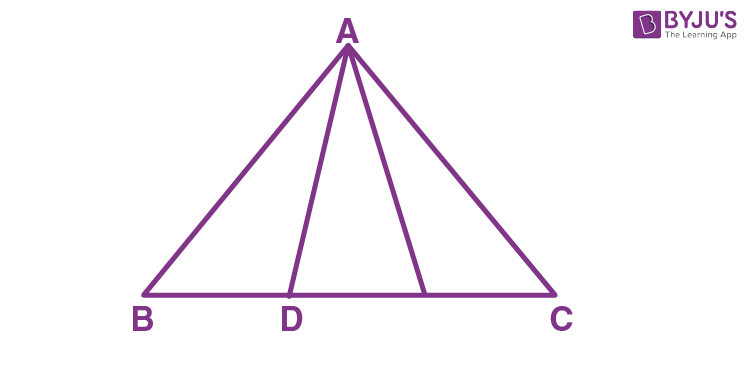In ΔABC, As BD = ½ DC, we have

BD/DC = ½

So, Ar(ΔABD): Ar(ΔADC) = 1: 2

But, Ar(ΔABD) + Ar(ΔADC) = Ar(ΔABC)

Ar(ΔABD) + 2 Ar(ΔABD) = Ar(ΔABC)

3 Ar(ΔABD) = Ar(ΔABC)

Thus,

Ar(ΔABD) = 1/3 Ar(ΔABC)

6. In a parallelogram ABCD, point P lies in DC such that DP: PC = 3: 2. If area of ΔDPB = 30 sq. cm, find the area of the parallelogram ABCD.

Solution:

We know that,

Ratio of area of triangles with same vertex and bases along the same line is equal to ratio of their respective bases.

So, we have

Area (ΔDPB)/Area (ΔPCB) = DP/PC = 3/2

Given, area of ΔDPB = 30 sq. cm

Let ‘x’ be the area of ΔPCB,

Then,

30/x = 3/2

x = 30/3 x 2 = 20 sq. cm.

Therefore, area of ΔPCB = 20 sq. cm

Now, consider the following figure.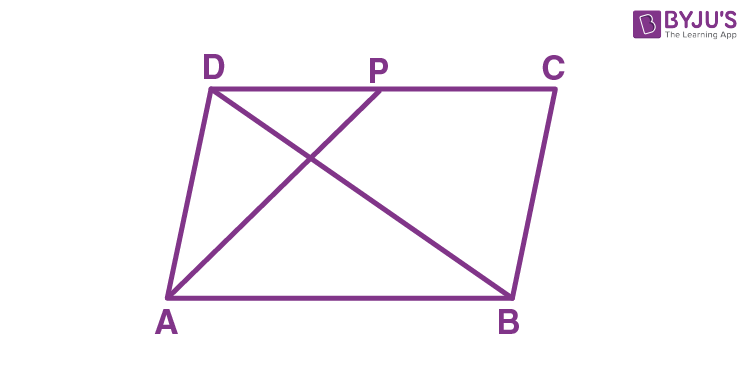It is seen clearly from the diagram,

Area (ΔCDB) = Area (ΔDPB) + Area (ΔCPB)

= 30 + 20

= 50 sq. cm

Diagonal of the parallelogram divides it into two triangles ΔADB and ΔCDB of equal area.

Therefore,

Area (||gm ABCD) = 2 x ΔCDB

= 2 x 50

= 100 sq. cm

7. ABCD is a parallelogram in which BC is produced to E such that CE = BC and AE intersects CD at F. If ar. (ΔDFB) = 30 cm2; find the area of parallelogram.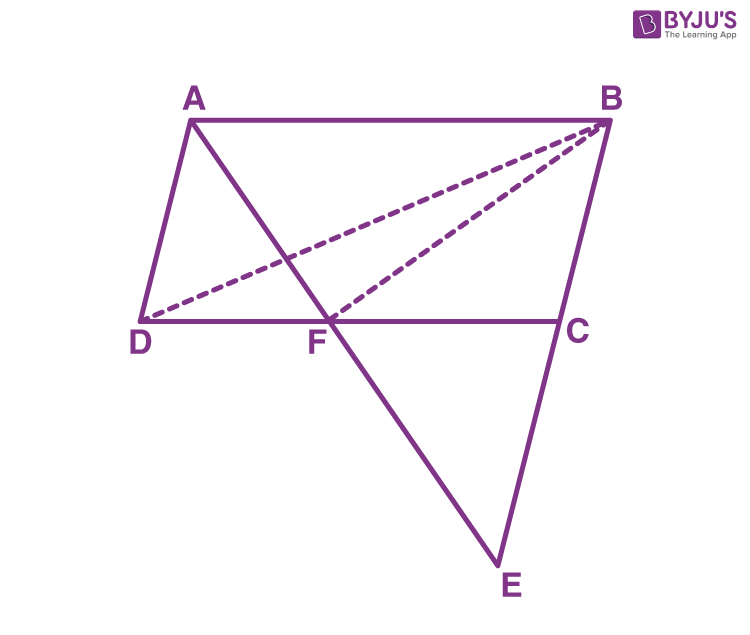Solution:

Given, BC = CE

Also, in ||gm ABCD we have

In ΔADF and ΔECF, we have

∠DAF = ∠CEF (Alternate angles)

Thus, ΔADF ≅ ΔECF by ASA congruence criterion

So, area (ΔADF) = area (ΔECF) … (i)

Also,

In ΔFBE, FC is the median (As BC = CE)

So, Area (ΔBCF) = Area (ΔECF) … (ii)

From (i) and (ii), we have

Area (ΔADF) = Area (ΔBCF) … (iii)

Again,

As ADF and BDF are on the same base DF and between the same parallels DF and AB

Area (ΔBDF) = Area (ΔADF) … (iv)

From (iii) and (iv), we have

Area (ΔBDF) = Area (ΔBCF)

Given, Area (ΔDFB) = 30 cm2

So, Area (ΔBCF) = 30 cm2

Area (ΔBCD) = Area (ΔBDF) + Area (ΔBCF)

= (30 + 30) cm2

= 60 cm2

Hence,

Area of ||gm ABCD = 2 x Area(ΔBCD)

= 2 x 60

= 120 cm2

8. The following figure shows a triangle ABC in which P, Q and R are mid-points of sides AB, BC and CA respectively. S is mid-point of PQ.

Prove that: ar.(∆ABC) = 8 × ar.(∆QSB)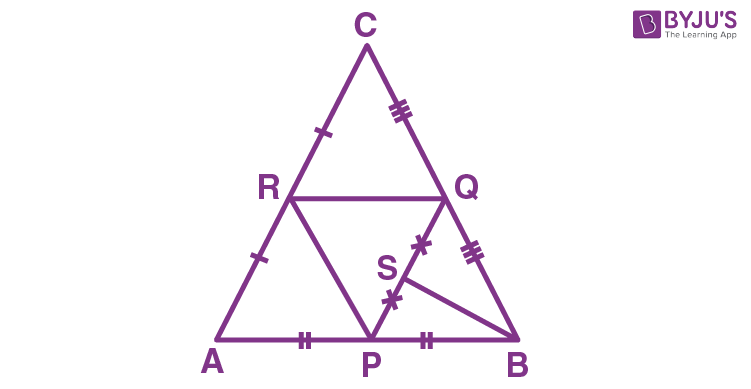Solution:

In ∆ABC, R and Q are the mid-points of AC and BC respectively

So, by mid-point theorem RQ || AB

⇒ RQ || PB

So, area(∆PBQ) area(∆APR) …(i) [Since AP = PB and triangles on the same base and between the same parallels are equal in area]

Now,

Since P and R are the mid-points of AB and AC respectively.

By mid-point theorem, PR || BC

⇒ PR || BQ

So, quadrilateral PMQR is a parallelogram.

Also, area(∆PBQ) = area(∆PQR) …(ii) [diagonal of a parallelogram divide the parallelogram in two triangles with equal area]

From (i) and (ii), we have

Area (∆PQR) = area(∆PBQ) = area(∆APR) … (iii)

Similarly, P and Q the mid-points of AB and BC respectively.

By mid-point theorem,

PQ || AC ⇒ PQ || RC

So, quadrilateral PQRC is a parallelogram.

Also, area(∆RQC) = area(∆PQR) …(iv) [Diagonal of parallelogram divide the parallelogram in two triangles with equal area]

From (iii) and (iv),

Area(∆PQR) = area(∆PBQ) = area(∆RQC) = area(∆APR)

So, area (∆PBQ) = ¼ area (∆ABC) …(v)

Also, since S is the mid-point of PQ

BS is the median of ∆PBQ

So, area (∆QSB) = ½ area(∆PBQ)

Now from (v), we have

Area(∆QSB) = ½ × ¼ area (∆ABC)

Area(∆ABC) = 8 × area(∆QSB)

Exercise 16(C)

1. In the given figure, the diagonals AC and BD intersect at point O. If OB = OD and AB//DC, show that:

(i) Area (Δ DOC) = Area (Δ AOB).

(ii) Area (Δ DCB) = Area (Δ ACB).

(iii) ABCD is a parallelogram.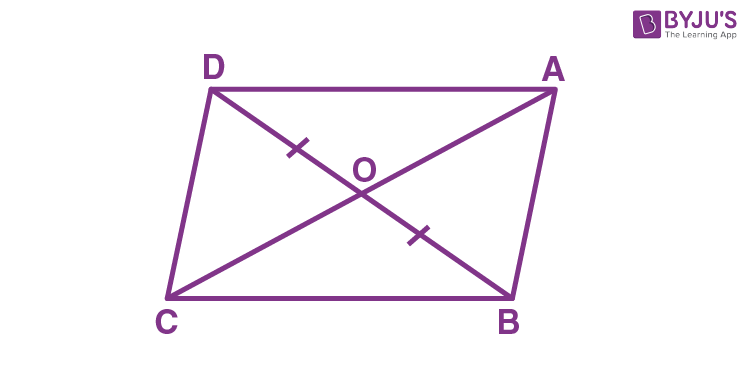Solution:

(i) Ratio of area of triangles with same vertex and bases along the same line is equal to the ratio of their respective bases.

So, we have

Area of ∆DOC/Area of ∆BOC = DO/BO = 1 … (i)

Similarly,

Area of ∆DOA/Area of ∆BOA = DO/BO = 1 … (ii)

We know that area of triangles on the same base and between same parallel lines are equal.

Area of Δ ACD = Area of Δ BCD

Area of Δ AOD + Area of Δ DOC = Area of Δ DOC + Area of Δ BOC

⇒ Area of Δ AOD = Area of Δ BOC … (iii)

From (i), (ii) and (iii) we have

Area (Δ DOC) = Area (Δ AOB)

– Hence Proved.

(ii) Similarly, from 1, 2 and 3, we also have

Area of Δ DCB = Area of Δ DOC + Area of Δ BOC = Area of Δ AOB + Area of Δ BOC = Area of Δ ABC

So, Area of Δ DCB = Area of Δ ABC

– Hence Proved.

(iii) We know that area of triangles on the same base and between same parallel lines are equal.

Given: triangles are equal in area on the common base, so it indicates AD|| BC.

So, ABCD is a parallelogram.

– Hence Proved

2. The given figure shows a parallelogram ABCD with area 324 sq. cm. P is a point in AB such that AP: PB = 1:2 Find The area of ΔAPD.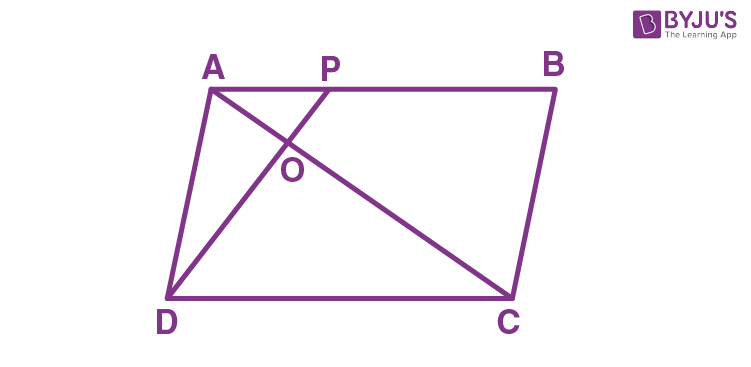Solution:

Ratio of area of triangles with the same vertex and bases along the same line is equal to the ratio of their respective bases.

So, we have

Area of ∆APD/Area of ∆BPD = AP/BP = ½

Area of parallelogram ABCD = 324 sq.cm

Area of the triangles with the same base and between the same parallels are equal.

We know that area of the triangle is half the area of the parallelogram if they lie on the same base and between the parallels.

Therefore, we have,

Area(∆ABD) = ½ × Area(||gm ABCD)

= 324/2

= 162 sq. cm

Also, from the diagram it is clear that

Area(∆ABD) = Area(∆APD) + Area(∆BPD)

162 = Area(∆APD) + 2 × Area(∆APD)

162 = 3 × Area(∆APD)

Area(∆APD) = 162/3

= 54 sq. cm

(ii) Consider ∆AOP and ∆COD

∠AOP = ∠COD [Vertically opposite angles]

∠CDO = ∠APD [AB || DC and DP is the transversal, alternate interior angles are equal]

Thus, ∆AOP ~ ∆COD by AA similarity

Hence the corresponding sides are proportional.

AP/CD = OP/OD

= AP/AB

= AP/(AP+PB)

= AP/3AP

= 1/3

Therefore, OP: OD = 1: 3

3. In ∆ABC, E and F are mid-points of sides AB and AC respectively. If BF and CE intersect each other at point O, prove that the ∆OBC and quadrilateral AEOF are equal in area.

Solution:

Given, E and F are the midpoints of the sides AB and AC

Let’s consider the following figure.Therefore, by midpoint theorem

We have, EF || BC

Triangles BEF and CEF lie on the common base EF and between the parallels, EF and BC

Therefore, Ar(∆BEF) = Ar(∆CEF)

⇒ Ar(∆BOE) + Ar(∆EOF) = Ar(∆EOF) + Ar(∆COF)

Ar(∆BOE) = Ar(∆COF)

Now, BF and CE are the medians of the triangle ABC

And, median of the triangle divide it into two equal areas of triangles.

Thus, Ar(∆ABF) = Ar(∆CBF)

Now, subtracting Ar(∆BOE) on the both the sides, we get

Ar(∆ABF) – Ar(∆BOE) = Ar(∆CBF) – Ar(∆BOE)

Since, Ar(∆BOE) = Ar(∆COF)

⇒ Ar(∆ABF) – Ar(∆BOE) = Ar(∆CBF) – Ar(∆COF)

– Hence proved

4. In parallelogram ABCD, P is mid-point of AB. CP and BD intersect each other at point O. If area of ∆POB = 40 cm2, and OP: OC = 1: 2, find:

(i) Areas of ∆BOC and ∆PBC

(ii) Areas of ∆ABC and parallelogram ABCD.

Solution:

(i) On joining AC, we have the following figure as below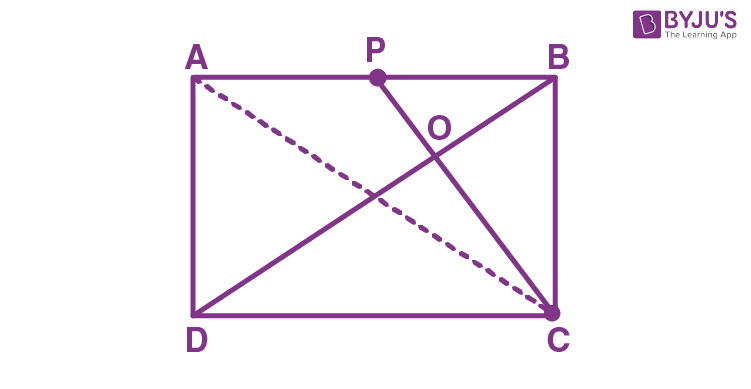Let’s consider ∆POB and ∆COD

∠POB = ∠DOC [Vertically opposite angles]

∠OPB = ∠ODC [Since, AB || DC; CP and BD are the transversal, alternate interior angles are equal]

Therefore, ∆POB ~ ∆COD by AA similarity criterion

As, P is the midpoint

AP = BP and AB = CD, we have CD = 2BP

Therefore, we have

BP/CD = OP/OC = OB/OD = ½

OP: OC = 1: 2

(ii) From (i), we have

BP/CD = OP/OC = OB/OD = ½,

Ratio between the areas of two similar triangles is equal to the ratio between the square of the corresponding sides

Here, ∆DOC and ∆POB are similar triangles.

Thus, we have

Ar(∆DOC)/Ar(∆POB) = DC2/PB2

Ar(∆DOC)/Ar(∆POB) = (2PB)2/PB2

Ar(∆DOC)/Ar(∆POB) = 4PB2/PB2

Ar(∆DOC)/Ar(∆POB) = 4

Ar(∆DOC) = 4 × Ar(∆POB)

= 4 × 40

=160 cm2

Now, consider Ar(∆DBC) = Ar(∆DOC) + Ar(BOC)

= 160 + 80

= 240 cm2

Two triangles are equal in area if they are on the equal bases and between the same parallels

Therefore,

Ar(∆DBC) = Ar(∆ABC) = 240 cm2

We know that,

Median divides the triangle into areas of two equal triangles

Thus, CP is the median of the triangle ABC.

Hence, Ar(∆ABC) = 2 × Ar(∆PBC)

Ar(∆PBC) = Ar(∆ABC)/2

= 240/2

= 120cm2

(iii) From (ii), we have

Ar(∆ABC) = 2 × Ar(∆PBC) 240 cm2

Area of a triangle is half the area of the parallelogram

If both are on equal bases and between the same parallels

Thus, Ar(∆ABC) = ½ Ar(||gm ABCD)

⇒ Ar(llgm ABCD) = 2 Ar(∆ABC)

= 2 × 240

= 480 cm2

5. The medians of a triangle ABC intersect each other at point G. If one of its medians is AD, prove that:

(i) Area (∆ABD) = 3 × Area (∆BGD)

(ii) Area (∆ACD) = 3 × Area (∆CGD)

(iii) Area (∆BGC) = 1/3 × Area (∆ABC)

Solution:

The figure is as shown below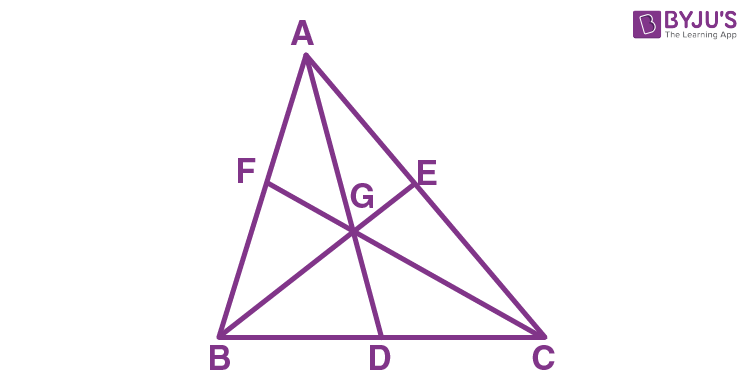We know that, medians interest at the centroid

Given that G is the point of intersect of medians

So, G is the centroid of the triangle ABC.

Now, the centroid divides the medians in the ratio 2:1

(i) We have, AG:GD = 2:1

So, Area(∆AGB)/Area(∆BGD) = 2/1

Area(∆AGB) = 2Area(∆BGD)

Now, from the figure, it is clearly seen that

Area(∆ABD) = Area(∆AGB) + Area(∆BGD)

Area(∆ABD) = 2 × Area(∆BGD) + Area(∆BGD)

Thus, Area(∆ABD) = 3 × Area(∆BGD) … (1)

(ii) Similarly, CG divides AD in the ratio 2:1

So, Area(∆AGC) / Area(∆CGD) = 2/1

Area(∆AGC) = 2 × Area(∆CGD)

Now, from the figure, it is clearly seen that

Area(∆ACD) = Area(∆AGC) + Area(∆CGD)

Area(∆ACD) = 2 × Area(∆CGD) + Area(∆CGD)

Thus, Area(∆ACD) = 3 × Area(∆CGD) … (2)

(iii) Adding equation (1) and (2), we have

Area(∆ABD) + Area(∆ACD) = 3Area(∆BGD) + 3Area(∆CGD)

Area(∆ABC) = 3 × [Area(∆BGD0 + Area(∆CGD)]

Area(∆ABC) = 3 × Area(∆BGC)

Area(∆ABC)/3 = Area(∆BGC)

Thus, Area(∆BGC) = 1/3 × Area(∆ABC)

6. The perimeter of a triangle ABC is 37 cm and the ratio between the lengths of its altitudes be 6 : 5 : 4. Find the lengths of its sides.

Solution:

Let’s consider the sides of ABC to be x cm, y cm and (37 – x – y) cm

Also, let the lengths of its altitudes be 6a cm, 5a cm and 4a cm

We know that,

Area of a triangle= ½ × base × altitude

⇒ ½ × 6a = ½ × y × 5a = ½ × (37 – x – y) × 4a

6x = 5y = 148 – 4x – 4y

6x = 5y and 6x = 148 – 4x – 4y

6x – 5y = 0 and 10x + 4y – 148 = 0

Now, by solving both the equations, we have

x = 10 cm and y = 12 cm

And, (37 – x – y) = 15cm

7. In parallelogram ABCD, E is a point in AB and DE meets diagonal AC at point F. If DF:FE = 5:3 and area of ∆ADF is 60 cm2; find

(ii) If AE:EB = 4:5, find the area of ∆ADB

(iii) Also, find area of parallelogram ABCD

Solution: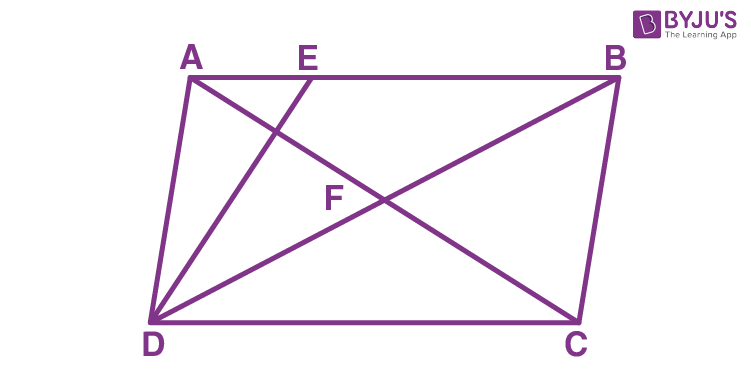Triangles ADF and AFE have the same vertex A and their bases are on the same straight line DE

60/Ar(∆AFE) = 5/3

Ar(∆AFE) = (60 × 3)/5

= 36 cm2

= 60 cm2 + 36 cm2

= 96 cm2

∆ADE and ∆EDB have their bases are on the same straight line AB

96/Ar(∆EDB) = 4/5

Ar(∆EDB) = (96 × 5)/4

= 120cm2

= 96 cm2 + 120 cm2

= 216 cm2

Now,

∆ADB and ||m ABCD are on the same base AB and between the same parallels AB and DC

∴ Ar(ADB) = ½ Ar(||m ABCD)

216 = ½ Ar(||m ABCD)

Thus, Ar(||m ABCD) = 2 × 216

= 432 cm2

8. In the following figure, BD is parallel to CA, E is mid-point of CA and BD = ½ CA.

Prove that: Ar (ABC) = 2 x Ar (DBC)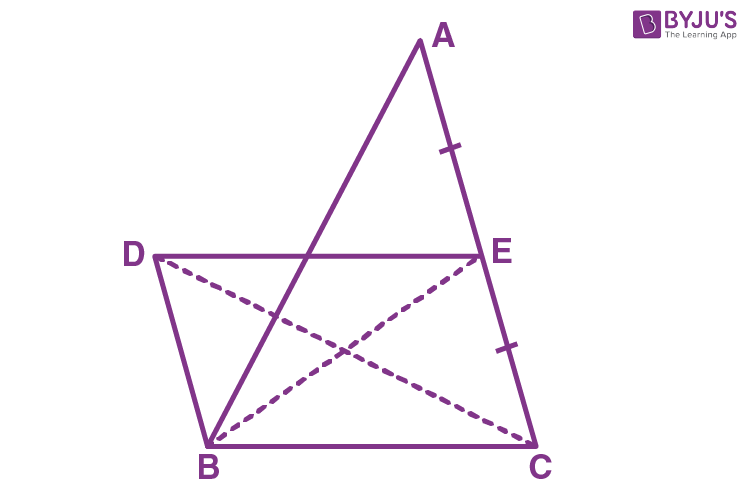Solution:

Here, BCED is a parallelogram, since BD = CE and BD || CE.

Now,

Ar(∆DBC) = Ar(∆EBC) … (i) [Since they have the same base and area between the same parallels]

In ∆ABC, we have

BE as the median

So, Ar(∆EBC) = ½ [Ar(∆EBC) + Ar(∆ABE)] = ½ Ar(∆ABC)

⇒ Ar(∆ABC) = 2Ar(∆EBC)

Therefore, Ar(∆ABC) = 2Ar(∆DBC) [From (i)]

9. In the following figure, OAB is a triangle and AB ∥ DC.If the area of ∆CAD = 140 cm2 and the area of ∆ODC = 172 cm2, find

(i) the area of ∆DBC

(ii) the area of ∆OAC

(iii) the area of ∆ODB.

Solution:

Given: ∆CAD = 140cm2, ∆ODC = 172cm2 and AB || CD

As triangles DBC and CAD have the same base CD and between the same parallel lines Hence,

= 140cm2 + 172cm2

= 312cm2

Ar(∆ODB) = Ar(∆DBC) + Ar(∆ODC)

= 140cm2 + 172cm2

= 312cm2

10. E, F, G and H are the mid- points of the sides of a parallelogram ABCD. Show that area of quadrilateral EFGH is half of the area of parallelogram ABCD.

Solution:

Let’s join HF.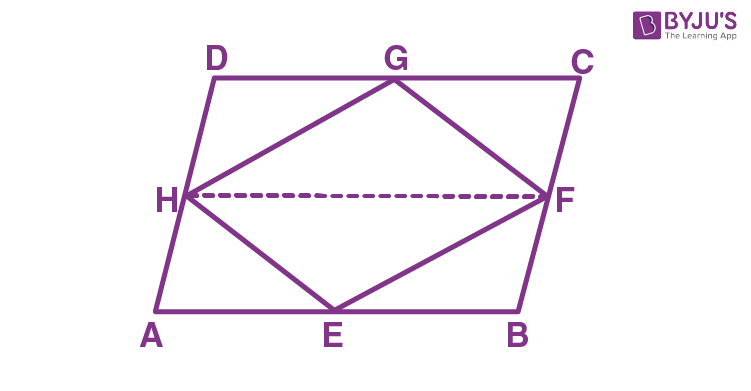Since H and F are mid-points of AD and BC respectively, we have

AH = ½ AD and BF = ½ BC

Now, ABCD is a parallelogram

So, AH = BF and AH ∥ BF

Thus, ABFH is a parallelogram

Now,

Since parallelogram FHAB and ΔFHE are on the same base FH and between the same parallels HF and AB, we have

Ar(∆FHE) = ½ A(∥mFHAB) …(i)

Similarly,

Ar(∆FHG) = ½ Ar(∥mFHDC) …(ii)

Adding (i) and (ii), we get

Ar(∆FHE) + Ar(∆FHG) = ½ Ar(∥mFHAB) + ½ Ar(∥mFHDC)

Ar(EFGH) = ½ [Ar(∥mFHAB) + ½ Ar(∥mFHDC)]

∴ Ar(EFGH) = ½ Ar(∥mABCD)

11. ABCD is a trapezium with AB parallel to DC. A line parallel to AC intersects AB at X and BC at Y. Prove that area of ∆ADX = area of ∆ACY.

Solution:

Let’s join CX, DX and AY.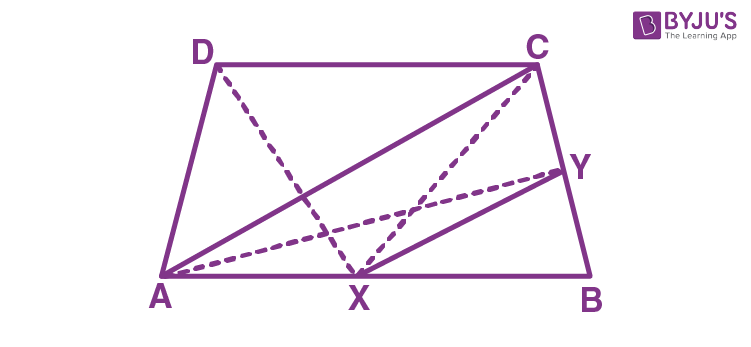Now, triangles ADX and ACX are on the same base AX and between the parallels AB and DC.

Also, triangles ACX and ACY are on the same base AC and between the parallels AC and XY.

∴ Ar(ΔACX) = Ar(ΔACY) ….(ii)

From (i) and (ii), we get

– Hence proved

## Selina Solutions for Class 9 Maths Chapter 16- Area Theorems

The Chapter 16, Area Theorems, contains 3 exercises and the Selina Solutions given here has the answers for all the questions present in these exercises. Let us have a look at some of the topics that are being discussed in this chapter.

16.1 Introduction

16.2 Figures between the same parallels

16.3 Triangles with the same vertex and bases along the same line

## Selina Solutions for Class 9 Maths Chapter 16- Area Theorems

Area of a plane is nothing but the region bounded by the plane figure. Students also know that equal figures mean, the figures equal in areas. The Chapter 16 of class 9 helps the students in understanding the different theorems related to the areas of different polygons. Read and learn the Chapter 16 of Selina textbook to learn more about Area Theorems along with the concepts covered in it. Solve the Selina Solutions for Class 9 effectively to score high in the examination.